https://doi.org/10.5194/acp-22-371-2022
https://doi.org/10.5194/acp-22-371-2022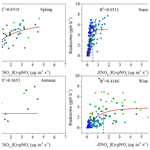# Exploration of the atmospheric chemistry of nitrous acid in a coastal city of southeastern China: results from measurements across four seasons

Baoye Hu, Jun Duan, Youwei Hong, Lingling Xu, Mengren Li, Yahui Bian, Min Qin, Wu Fang, Pinhua Xie, and Jinsheng Chen
Abstract

Because nitrous acid (HONO) photolysis is a key source of hydroxyl (OH) radicals, identifying the atmospheric sources of HONO is essential to enhance the understanding of atmospheric chemistry processes and improve the accuracy of simulation models. We performed seasonal field observations of HONO in a coastal city of southeastern China, along with measurements of trace gases, aerosol compositions, photolysis rate constants (J), and meteorological parameters. The results showed that the average observed concentration of HONO was 0.54 ± 0.47 ppb. Vehicle exhaust emissions contributed an average of 1.45 % to HONO, higher than the values found in most other studies, suggesting an influence from diesel vehicle emissions. The mean conversion frequency of NO2 to HONO in the nighttime was the highest in summer due to water droplets evaporating under high-temperature conditions. Based on a budget analysis, the rate of emission from unknown sources (Runknown) was highest around midday, with values of 4.51 ppb h−1 in summer, 3.51 ppb h−1 in spring, 3.28 ppb h−1 in autumn, and 2.08 ppb h−1 in winter. Unknown sources made up the largest proportion of all sources in summer (81.25 %), autumn (73.99 %), spring (70.87 %), and winter (59.28 %). The photolysis of particulate nitrate was probably a source in spring and summer while the conversion from NO2 to HONO on BC enhanced by light was perhaps a source in autumn and winter. The variation of HONO at night can be exactly simulated based on the HONO $/$ NOx ratio, while the J(NO${}_{\mathrm{3}}^{-}$_R) ×pNO${}_{\mathrm{3}}^{-}$ should be considered for daytime simulations in summer and autumn, or $\mathrm{1}/\mathrm{4}×$ (J(NO${}_{\mathrm{3}}^{-}$_R) ×pNO${}_{\mathrm{3}}^{-}$) in spring and winter. Compared with O3 photolysis, HONO photolysis has long been an important source of OH except for summer afternoons. Observation of HONO across four seasons with various auxiliary parameters improves the comprehension of HONO chemistry in southeastern coastal China.

Share
Dates
1 Introduction

Nitrous acid (HONO) photolysis produces hydroxyl radical (OH), an important oxidant, in the troposphere (Zhou et al., 2011). OH plays an important role in triggering the oxidation of volatile organic compounds and therefore determines the fate of many anthropogenic atmospheric pollutants (Lei et al., 2018). Recent research results have shown that HONO production is the cause of an increase in secondary pollutants (Li et al., 2010; Gil et al., 2019; Fu et al., 2019). Though extensive studies have been conducted in the four decades since the first clear measurement of HONO (Perner and Platt, 1979), the HONO formation mechanisms are still elusive, especially during the daytime, when there is a large difference between measured concentrations and those calculated from known gas-phase chemistry (Sörgel et al., 2011). Identification of the sources of atmospheric HONO and exploration of its formation mechanisms are beneficial for enhancing our comprehension of atmospheric chemistry processes and improving the accuracy of atmospheric simulation models.

Commonly accepted HONO sources include direct emission from motor vehicles (Chang et al., 2016; Kirchstetter et al., 1996; Kramer et al., 2020; Xu et al., 2015) or soil (Su et al., 2011; Tang et al., 2019; Oswald et al., 2013), the homogeneous conversion of NO by OH (Seinfeld and Pandis, 1998; Kleffmann, 2007), and the heterogeneous reaction of NO2 on humid surfaces (Alicke, 2002; Finlayson-Pitts et al., 2003). Other homogeneous sources include nucleation reactions of NH3, NO2, and H2O (Zhang and Tao, 2010); electronically excited H2O and NO2 for the production of HONO (Li et al., 2008); and the HO2 H2O complex and NO2 for the production of HONO (Li et al., 2014). Other heterogeneous sources include NO2 reduced on soot to produce HONO and drastically enhanced by light (Ammann et al., 1998; Monge et al., 2010), semivolatile organics from diesel exhaust for the production of HONO (Gutzwiller et al., 2002), photoactivation of NO2 on humic acid (Stemmler et al., 2006), TiO2 (Ndour et al., 2008), solid organic compounds (George et al., 2005), the photolysis of particulate nitrate by ultraviolet (UV) light (Kasibhatla et al., 2018; Romer et al., 2018; Ye et al., 2017; Scharko et al., 2014), dissolution of NO2 catalyzed by anions on aqueous microdroplets (Yabushita et al., 2009), the process of acid displacement (Vandenboer et al., 2014), the conversion of NO2 to HONO on the ground (Wong et al., 2011), NH3 enhancing the heterogeneous reaction of NO2 with SO2 for the production of HONO (Ge et al., 2019), NH3 promoting NO2 dimer hydrolysis for HONO production through stabilizing the state of the product and reducing the reaction free energy barrier (L. Li et al., 2018; Xu et al., 2019), and heterogeneous formation of HONO catalyzed by CO2 (Xia et al., 2021). Heterogeneous processes are the most poorly understood and yet are widely considered the main sources of HONO in previous studies. The uptake coefficients of NO2 conversion to HONO on surfaces (including aerosols, ground, buildings, and vegetation) vary from 10−9 to 10−2, derived from different experiments (Ammann et al., 1998; Kirchner et al., 2000; Underwood et al., 2001; Aubin and Abbatt, 2007; Zhou et al., 2015; Liu et al., 2014; Vandenboer et al., 2013). It is still a challenge to extrapolate laboratory results to real surfaces. There is still research being carried out to distinguish the key step to determine the NO2 uptake, and we are also not sure what role radiation plays in it. The absence of major HONO sources during the daytime is another subject of active ongoing research.

According to an analysis of 15 sets of field observations around the world (Elshorbany et al., 2012), the HONO $/$ NOx ratio (0.02) predicts well HONO concentrations under different atmospheric conditions. To avoid underestimation of HONO in this study, an empirical parameterization was applied to estimate the HONO concentration, because the current understanding of HONO formation mechanisms is incomplete. Field measurements of HONO and its precursor NO2 at sites with different aerosol load and composition, photolysis rate constants, and meteorological parameters are necessary to deepen our knowledge of the HONO formation mechanisms. Such measurements have been carried out in coastal cities in China, including Guangzhou (Qin et al., 2009), Hong Kong (Xu et al., 2015), and Shanghai (Cui et al., 2018), where the air pollution was relatively severe during the research period. However, there has been a lack of research into HONO in coastal cities with good air quality and low concentrations of PM2.5, but strong sunlight and high humidity. Insufficient research on coastal cities with good air quality has resulted in certain obstacles to assessing the photochemical processes in these areas. Due to different emission-source intensities and ground surfaces, the atmospheric chemistry of HONO in the southeastern coastal area of China is predicted to have different pollution characteristics from those found in other coastal cities. Furthermore, HONO contributes to the atmospheric photochemistry differently depending on the season (Li et al., 2010). Therefore, observations of atmospheric HONO across different seasons in the southeastern coastal area of China are urgently needed.

Incoherent broadband cavity-enhanced absorption spectroscopy (IBBCEAS) was employed in this study to determine HONO concentrations in the southeastern coastal city of Xiamen in August (summer), October (autumn), and December (winter) 2018 and March (spring) 2019. In addition, a series of other relevant trace gases, meteorological parameters, and photolysis rate constants were measured at the same time to provide additional information to reveal the HONO formation mechanisms. The main purposes of this study were to (1) calculate the values of unknown HONO daytime sources, (2) analyze the processes leading to HONO formation, (3) simulate HONO concentrations based on an empirical parameterization, and (4) evaluate OH production from HONO from 07:00 to 16:00 local time (LT). These results were compared between the seasons.

2 Methodology

## 2.1 Site description

Our field observations were carried out  80 m above the ground at a supersite located on the top of the Administrative Building of the Institute of Urban Environment (IUE), Chinese Academy of Sciences (1180413′′ E, 24 3652′′ N), in Xiamen, China, in August, October, and December 2018 and March 2019 (Fig. 1). The supersite was equipped with a complete set of measurement tools, including those for measuring gases and aerosol species composition, meteorology parameters, and photolysis rate constants, which provided a good chance to study the atmospheric chemistry of HONO in a coastal city of southeastern China. As shown in Fig. 1 (left), Xiamen is located at the southeastern coastal area of China and faces the Taiwan Strait in the east. It suffers from sea and land breeze throughout the year with spring and summer more frequently (Xun et al., 2017). The IUE supersite is surrounded by Xinglin Bay, several universities (or institutes), and several major roads with a large traffic fleet, such as Jimei Road, Shenhai Expressway (870 m), and Xiasha Expressway (2300 m) (Fig. 1 (right)). The area of Xiamen is 1700.61 km2 with a population of 4.11 million (http://tjj.xm.gov.cn/tjzl/, last access: 12 August 2019). The number of motor vehicles in 2018 was 1 572 088, which was 2.73 times as many as 10 years ago. The surrounding soil is used for landscape greening, not for agricultural production.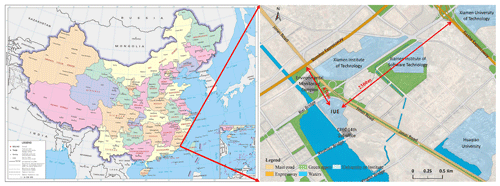Figure 1Location of Xiamen in China (left) and surroundings of IUE (right). Note: the map on the left was directly downloaded from http://bzdt.ch.mnr.gov.cn/ (last access: 22 September 2021), while the map in the right was significantly enriched based on a layer download from http://www.rivermap.cn/ (last access: 25 October 2020). The copyright statement of Fig. 1 on the left is © 2021 SinoMaps Press and National Geomatics Center of China.

## 2.2 Instrumentation

The atmospheric concentrations of both HONO and NO2 were determined using IBBCEAS, which has previously been widely applied to such measurements (Tang et al., 2019; Duan et al., 2018; Min et al., 2016). The IBBCEAS instrument was customized by the Anhui Institute of Optics and Fine Mechanics (AIOFM), Chinese Academy of Sciences (Duan et al., 2018). The resonant cavity is composed of a pair of highly reflective mirrors separated by 70 cm, and their reflectivity is approximately 0.99983 at 368.2 nm. The surface of the mirrors was purged by dry nitrogen at 0.1 standard liters per minute (SLM), and the air flow was controlled by a mass flow controller to prevent the surface of the mirror from being contaminated. Light was introduced into the resonant cavity and was emitted by a single light-emitting diode (LED) with full width at half maximum (FWHM) of 13 nm and a peak wavelength of 365 nm. Light transmitted through the cavity was received by a spectrometer (QE65000, Ocean Optics Inc., USA) through an optical fiber with 600 µm diameter and a 0.22 numerical aperture.

In order to avoid the drift of the center wavelength of the LED, the temperature of the LED was controlled to be approximately 25 ± 0.01 C by using a thermoelectric cooler unit. In order to prevent particulate matter from entering the cavity and reducing the effect of particulate matter on the effective absorption path, a 1 µm polytetrafluoroethylene (PTFE) filter membrane (Tisch Scientific) was used in the front end of the sampling port. In order to ensure the quality of the data, the 1 µm PTFE filter membrane was usually replaced once every three days and the sampling tube was thoroughly cleaned with alcohol once a month. We increased the replacement frequency of the filter membrane and the cleaning frequency of the sampling tube in the event of heavy pollution to ensure that the filter membrane and sampling tube are in a clean state. The length of sampling tube with 6 mm outer diameter was approximately 3 m, the material was PFA with excellent chemical inertness, and the sampling flow rate was 6 SLM meaning that the residence time of the gas in the sampling tube was less than 0.5 s. Besides, the sampling loss was calibrated before the experiment. We assessed the measured spectrum every day to ensure the authenticity of the measurement results. Multiple reflections in the resonator cavity enhanced the length of the effective absorption path, thereby enhancing the detection sensitivity of the instrument. The 1σ detection limits for HONO and NO2 were about 60 and 100 ppt, respectively, and the time resolution was 1 min. The fitting wavelength range was selected as 359–387 nm. The measurement error of HONO of IBBCEAS was estimated to be about 9 %, considering both HONO secondary formation and sample loss. The sampling tube was heated to 35 C and covered by insulation cotton materials to prevent the effect of condensation of the water vapor (Lee et al., 2013).

The inorganic composition of PM2.5 aerosols (SO${}_{\mathrm{4}}^{\mathrm{2}-}$, NO${}_{\mathrm{3}}^{-}$, Cl, Na+, NH${}_{\mathrm{4}}^{+}$, K+, Ca2+, and Mg2+) and concentrations of gases (HONO, HNO3, HCl, SO2, NH3) were determined using a Monitor for AeRosols and Gases in ambient Air (MARGA, Model ADI 2080, Applikon Analytical B.V., the Netherlands). Ambient air was drawn into the sample box by a PM2.5 cyclone (Teflon coated, URG-2000-30ENB) at the flow rate of 1 m3 h−1. Air sample was drawn firstly through the wet rotating denuder (WRD) where gases diffused to the solution, and then particles were collected by a steam jet aerosol collector (SJAC). Absorption solutions were drawn from the SJAC and the WRD to syringes (25 mL). Samples were injected into Metrohm cation (500 µL loop) and anion (250 µL loop) chromatographs with the internal standard (LiBr) for 15 min after an hour when the syringes had been filled (Makkonen et al., 2012). Specific descriptions of the SJAC can be found in previous reports (Slanina et al., 2001; Wyers et al., 1993). Therefore, the times needed for the sampling period and the latter IC analysis on the MARGA system are a full hour and 15 min, respectively. The value measured in this hour is actually the concentration sampled in the previous hour, so the time corresponding to the sampling is matched with other instrument parameters (i.e., HONO, NOx, J values).

Photolysis frequencies were determined using a photolysis spectrometer (PFS-100, Focused Photonics Inc., Hangzhou, China). These were calculated by multiplying the actinic flux F, quantum yield φ(λ) and the known absorption cross section σ(φ). The measurements included the photolysis rate constants J (O1D), J (HCHO_M), J (HCHO_R), J(NO2), J (H2O2), J (HONO), J (NO3_M), and J (NO3_R), and the spectral band ranged from 270 to 790 nm. Hemispherical (2π sr) angular response deviations were within ±5 %. The photolysis rate constants with _R and _M represented a radical photolysis channel and molecular photolysis channel, respectively. Specifically, HCHO was removed by the Reactions (R1) and (R2), and NO3 was removed by the Reactions (R3) and (R4), respectively (Röckmann et al., 2010).

$\begin{array}{}\text{(R1)}& \mathrm{HCHO}+hv⟶\mathrm{CHO}+\mathrm{H}\phantom{\rule{1em}{0ex}}J\left(\mathrm{HCHO}\mathrm{_}\mathrm{R}\right)\text{(R2)}& \mathrm{HCHO}+hv⟶{\mathrm{H}}_{\mathrm{2}}+\mathrm{CO}\phantom{\rule{1em}{0ex}}J\left(\mathrm{HCHO}\mathrm{_}\mathrm{M}\right)\text{(R3)}& {\mathrm{NO}}_{\mathrm{3}}+hv⟶{\mathrm{NO}}_{\mathrm{2}}+{\mathrm{O}}^{\mathrm{3}}\mathrm{P}\phantom{\rule{1em}{0ex}}J\left({\mathrm{NO}}_{\mathrm{3}}\mathrm{_}\mathrm{R}\right)\text{(R4)}& {\mathrm{NO}}_{\mathrm{3}}+hv⟶\mathrm{NO}+{\mathrm{O}}_{\mathrm{2}}\phantom{\rule{1em}{0ex}}J\left({\mathrm{NO}}_{\mathrm{3}}\mathit{_}\mathrm{M}\right)\end{array}$

The O3 concentration was determined by an ultraviolet photometric analyzer (model 49i, Thermo Environmental Instruments (TEI) Inc.), and the limit of the instrument is 1.0 ppb. The NO concentration was determined by a chemiluminescence analyzer (TEI model 42i) with a molybdenum converter. The detection limit and the uncertainty of the TEI model 42i were 0.5 ppb and 10 %, respectively. Although the TEI model 42i also measures the concentration of NO2, this value might actually include other active nitrogen components (Villena et al., 2012). As expected, the NO2 concentration measured by IBBCEAS had the same trend as the NO2 measured by TEI 42i, and NO2 concentration measured by IBBCEAS was always lower than that by TEI 42i (Supplement Fig. S1). Therefore, the NO2 concentration as measured by IBBCEAS was used in this study. An oscillating microbalance with a tapered element was applied to determine the PM2.5 concentration with uncertainty of 10 %–20 %. Black carbon (BC) was measured using an Aethalometer at 7 wavelengths (in using 880 nm wavelength). When the tape was < 10 %, aethalometer fiber tape was replaced. Meteorological parameters were determined by an ultrasonic atmospherium (150WX, Airmar, USA). The time resolution of all instruments was unified to 1 h to facilitate comparison. Ultraviolet radiation (UV) was determined by a UV radiometer (Kipp & Zonen, SUV5 Smart UV Radiometer).

3 Results and discussion

## 3.1 Overview of data

Fig. 2 showed an overview of the determined HONO, NO, NO2, PM2.5, NO${}_{\mathrm{3}}^{-}$, BC, J(HONO), temperature (T), and relative humidity (RH) in this study. The entire campaign was characterized by a subtropical monsoon climate with high temperatures (9.82–34.42 C) and high humidity (29.24 %–100 %). The mean values (± standard deviation) of temperature and relative humidity were 22.24 ± 5.41 C and 78.35 ± 14.07 %, respectively. Elevated concentrations of NOx, i.e., up to 156.17 ppb of NO and 172.42 ppb of NO2, were observed, possibly due to dense vehicle emissions near this site. The photolysis rate constants J(O1D), J(HCHO_M), J(HCHO_R), J(NO2), J(H2O2), J(HONO), J(NO3_M), and J(NO3_R) had the same temporal variation (Fig. S2), although their orders of magnitude were different. The correlation coefficients between J(HONO) and other photolysis rate constants were above 0.965 (not shown). Both J(HONO) and UV peaked around noon, and the maximum of J(HONO) (2.02 × 10−3 s−1) and UV (55.62 W m−2) appeared at 13:00 LT on 11 March 2019, and 12:00 LT on 14 August 2018, respectively. This area was dominated by photochemical pollution, while particulate pollution was relatively light. No haze episodes occurred across four seasons with 111 d, because daily mass concentration of PM2.5 was lower than the National Ambient Air Quality Standard (Class II: 75 µg m−3). For O3, 10 episodes occurred with 8 h maximum concentrations of O3 exceeding the Class II: 160 µg m−3. Maximum mixing ratio of O3 was 113.81 ppb, occurring in the afternoon with strong ultraviolet radiation (42.72 W m−2) and a low NO concentration (0.75 ppb) of titrating O3. In general, the level of pollutants in this area was relatively low. Campaign-averaged levels of NO2, NO, NO${}_{\mathrm{3}}^{-}$, PM2.5, O3, and BC were 14.99 ± 8.93 ppb, 5.80 ± 11.98 ppb, 5.59 ± 6.26 µg m−3, 27.78 ± 17.95 µg m−3, 28.29 ± 21.14 ppb, and 1.67 ± 0.97 µg m−3, respectively. The maximum value of HONO (3.51 ppb) appeared at 08:00 LT on 4 December 2018. The high value of HONO was always accompanied by relative high values of NO and NO2 or PM2.5, BC, and NO${}_{\mathrm{3}}^{-}$. The average measured ambient HONO concentration at the measurement site for all measurement periods was 0.54 ± 0.47 ppb. The HONO concentration measured at this site was comparable to those measured at other suburban sites (Liu et al., 2019a; Xu et al., 2015; Nie et al., 2015; Park et al., 2004), was obviously lower than those measured at urban sites and industrial site (D. Li et al., 2018; Yu et al., 2009; Hou et al., 2016; Qin et al., 2009; Wang et al., 2013; Shi et al., 2020; Spataro et al., 2013; Huang et al., 2017; Wang et al., 2017), and was obviously higher than those measured at a marine background (Wen et al., 2019), marine boundary layer (Ye et al., 2016), and remote coastal region (Meusel et al., 2016), as shown in Table S1 in the Supplement.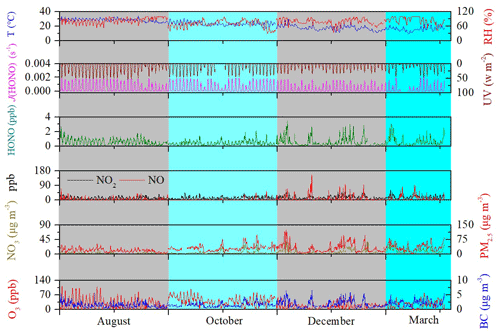Figure 2Time series of relative humidity (RH), temperature (T), J(HONO), UV, HONO, NO2, NO, NO${}_{\mathrm{3}}^{-}$, PM2.5, O3, and black carbon (BC) in Xiamen, China, in August, October, and December 2018 and March 2019. The missing data are mainly due to instrument maintenance.

As shown in Table 1, in the daytime (06:00–18:00 LT, including 06:00 LT), the highest concentration of HONO was found in spring and summer (0.72 ppb), followed by winter (0.61 ppb) and autumn (0.50 ppb). In short, the seasonal variation of HONO was well correlated with the seasonality of RH, with high RH in spring (84.21 %) and summer (84.12 %), followed by winter (78.13 %) and autumn (69.55 %). In conditions of low RH, the adsorption rate of NO2 is not as rapid as that of HONO, resulting in a reduction in the conversion rate of NO2 to HONO and thus a reduction in the concentration of HONO (Stutz et al., 2004). This seasonal variation in HONO concentration was different from those measured in Jinan (D. Li et al., 2018), Nanjing (Liu et al., 2019a), and Hong Kong (Xu et al., 2015). The elevated HONO concentrations in summer, when there is strong solar radiation, suggests the existence of strong sources of HONO and its important contribution to the production of OH radicals. Interestingly, the HONO concentration in the nighttime was lower than that in the daytime in all four seasons. Similar results were found in Hong Kong, which is also a coastal city (Xu et al., 2015). However, most previous studies have found that the HONO concentration at night is significantly higher than that during the day (Wang et al., 2015; Liu et al., 2019a; D. Li et al., 2018; Elshorbany et al., 2009; Acker et al., 2006; Yu et al., 2009). The higher HONO in the daytime is likely due to the higher NOx or nitrate photolysis as discussed in following section.

Table 1Overview of the HONO and NOx average concentrations measured in Xiamen and comparison with other measurements.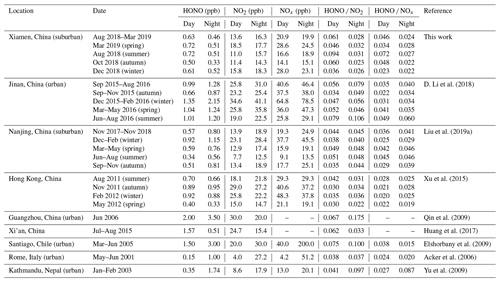Note: night (18:00–06:00 LT, including 18:00 LT); day (06:00–18:00 LT, including 06:00 LT). NOx= NO2 (IBBCEAS) + NO (Thermal 42i). IBBCEAS measures both HONO and NO2. The NO2 concentration is always overestimated by the Thermo Fisher 42i

.

The ratio of HONO to NOx or the ratio of HONO to NO2 have been extensively applied to indicate heterogeneous conversion of NO2 to HONO (Li et al., 2012; Liu et al., 2019a; Zheng et al., 2020). Compared with the HONO $/$ NO2 ratio, the HONO $/$ NOx ratio can better avoid the influence of primary emissions (Liu et al., 2019a). In this study, the HONO $/$ NOx ratios during the day were higher than those during the night, indicating that light promotes the conversion of NOx to HONO. The highest daytime HONO $/$ NOx ratio was found in summer (0.072), followed in turn by autumn (0.048), spring (0.034), and winter (0.023). The elevated HONO $/$ NOx ratio in summer indicates a greater net HONO production (Xu et al., 2015). The low HONO $/$ NOx ratio in winter can probably be ascribed to heavy emissions and high concentrations of NO in winter (Table 1). The HONO $/$ NOx ratios during every season in Xiamen were in general higher than those found in studies of other cities, which indicates greater net HONO production in Xiamen.

The diurnal patterns of HONO, NOx, HONO $/$ NOx, and J(NO2) averaged for every hour in each season are shown in Fig. 3. As shown in Fig. 3a, the HONO concentration had similar diurnal variation patterns across the four seasons. The maximum values of the HONO concentration were 1.12 ppb in winter, 1.03 ppb in summer, 0.98 ppb in spring, and 0.65 ppb in autumn, and these occurred in the morning rush hour (07:00–08:00 LT), which indicates that direct vehicle emissions may be a significant source of HONO. The contribution of direct vehicle emissions to HONO will be quantified in Sect. 3.2. The HONO concentration reduced rapidly from the morning rush hour to sunset, and this was caused by rapid photolysis combined with increased height of the boundary layer. The minimum values of HONO concentration were 0.47 ppb in spring, 0.23 ppb in winter, 0.21 ppb in summer, and 0.14 ppb in autumn, and these appeared at sunset, between 16:00 and 18:00 LT. The HONO concentration increased gradually after sunset, which indicates that release from HONO sources exceeded its dry deposition (Wang et al., 2017). There was a slight difference in the diurnal variation of HONO between autumn and the other seasons. A rapid reduction of HONO after the morning rush hour was found in spring, summer, and winter. In comparison, the HONO in autumn had an almost constant concentration between 07:00 and 11:00 LT because NOx decreased slowly during this period.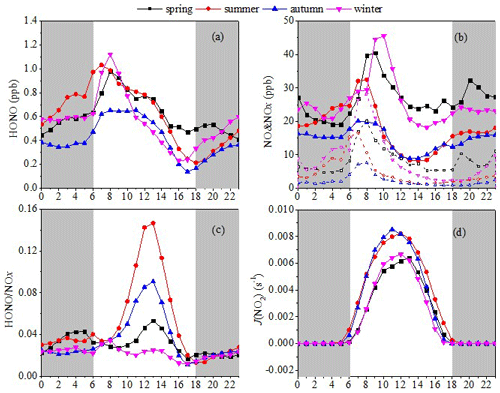Figure 3Diurnal variations in (a) HONO, (b) NO (hollow markers and dashed lines) and NOx (solid markers/lines), (c) HONO $/$ NOx, and (d) J(NO2). The gray shading indicates nighttime (18:00–06:00 LT, including 18:00 LT).

As shown in Fig. 3b, NOx concentration followed an expected profile in the four seasons, with peaks of 45.58 ppb in winter, 40.47 ppb in spring, 32.47 ppb in summer, and 20.07 ppb in autumn, each occurring in the morning rush hour at 10:00, 09:00, 08:00, and 07:00 LT, respectively. After these peaks, NOx decreased during the day in each season, probably due to photochemical transformation and increasing boundary-layer depth. The NOx concentrations then began to rise from their minima of 8.20 ppb in summer, 8.85 ppb in autumn, 18.10 ppb in winter, and 23.09 ppb in spring after 14:00, 13:00, 15:00, and 16:00 LT, respectively, which was caused by a combination of weak photochemical transformation and reduction in the boundary-layer depth. The NOx concentrations during winter and spring were significantly higher than those during autumn and summer. Both the maxima and minima of NOx appeared later in spring and winter compared with summer and autumn.

It is possible to better describe the behavior of HONO using the HONO $/$ NOx ratio. The higher HONO $/$ NOx ratio found at noon in the different seasons, especially in summer and autumn (Fig. 3c), indicates an additional daytime HONO source (Liu et al., 2019a; Xu et al., 2015). It is worth noting that the maximum value of this ratio in summer (0.147) was significantly higher than the maximum in other seasons, especially in winter (0.034). Figure 3d shows that the value of the HONO $/$ NOx ratio increased with the photolysis rate constant of NO2 in summer and autumn, suggesting that the additional HONO source is probably correlated with light (Xu et al., 2015; Wang et al., 2017; D. Li et al., 2018; Li et al., 2012). The increase in the HONO $/$ NO2 ratio during the day can be seen more clearly in Fig. 4, and its high value indicates a high HONO production efficiency, which cannot be ascribed to NO2 conversion due to the weak correlation between HONO and NO2 in summer. Furthermore, high HONO $/$ NO2 ratios were accompanied by high J(NO2) in summer, which indicates that HONO formation during the daytime is more likely to relate to light rather than Reaction (R5).

$\begin{array}{}\text{(R5)}& {\mathrm{NO}}_{\mathrm{2}}+{\mathrm{NO}}_{\mathrm{2}}+{\mathrm{H}}_{\mathrm{2}}\mathrm{O}\stackrel{\mathrm{surf}}{⟶}\mathrm{HONO}+{\mathrm{HNO}}_{\mathrm{3}}\end{array}$

However, the observed maxima can also be ascribed to sources independent from NOx concentration, such as soil emissions (Su et al., 2011) and photolysis of particulate nitrate (Zhou et al., 2011; Ye et al., 2016), which are not influenced by the decrease in NOx concentration around noon. A more specific discussion of daytime HONO sources considering the photolysis of particulate nitrate will be given in Sect. 3.4.3. The HONO emissions from soil were estimated to be 2–5 ppb h−1 (Su et al., 2011). However, soil emission was a negligible source of HONO in this study since the surrounding soil is not used for agriculture, and this greatly reduces the amount of HONO released due to the lack of a fertilization process (Su et al., 2011).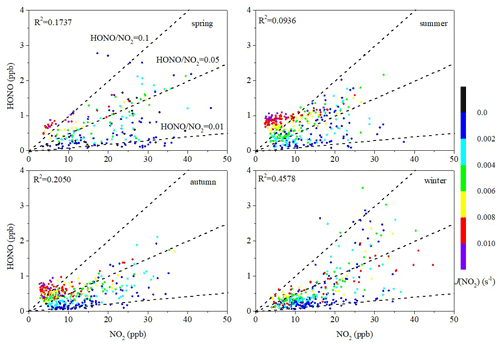Figure 4Scatter plots of NO2 versus HONO color coded by J(NO2). The three dashed lines represent 10 %, 5 %, and 1 % ratios of HONO $/$ NO2. Daytime was 06:00–18:00 LT, including 06:00 LT.

## 3.2 Direct vehicle emission of HONO

The K+ levels were 0.26, 0.13, 0.14, and 0.24 µg m−3 for spring, summer, autumn, and winter, respectively. The K+ levels during the four seasons were lower than 2 µg m−3, which indicated that biomass burning has little effect on this site (Xu et al., 2019). Hence, only vehicle emissions were considered in this study. The consistent diurnal variations in HONO and NOx presented in Sect. 3.1 (Fig. 3) also indicate HONO emissions from local traffic. Five criteria were applied to choose cases that guaranteed the presence of fresh plumes (Xu et al., 2015; Liu et al., 2019a): (1) UV < 10 W m−2; (2) short-duration air masses (< 2 h); (3) HONO correlating well with NOx (R2> 0.60, P< 0.05); (4) NOx> 20 ppb (highest 25 % of NOx value); and (5) ΔNO $/$ΔNOx> 0.85. A total of 23 cases met these strict criteria for estimation of the HONO vehicle emission ratios. The slopes of scatter plots of HONO vs. NOx were used as the emission factors.

A total of 23 vehicle emission plumes were summarized in Table 2, and these were used for estimation of the vehicle emission ratios. These plumes were considered to be truly fresh because the mean ΔNO $/$ΔNOx ratio (the linear slope of NO with NOx) of the selected air masses was 99 %. Vehicle plumes unavoidably mixing with other air masses resulted in the correlation coefficients (R2) between HONO and NOx varying among the cases, and these ranged from 0.64 to 0.92. The obtained ΔHONO $/$ΔNOx ratios (the linear slope of HONO with NOx) ranged from 0.24 % to 2.95 %, with an average value (±SD) of (1.45 ± 0.78) %. These ΔHONO $/$ΔNOx ratios have comparability to those obtained in Guangzhou (1.4 %, Qin et al., 2009; 1.8 %, Li et al., 2012) and Houston (1.7 %, Rappenglück et al., 2013) but are significantly higher than those measured in Jinan (0.53 %, D. Li et al., 2018) and Santiago (0.8 %, Elshorbany et al., 2009). The types of vehicle engine, the use of catalytic converters, and different fuels will affect the vehicle emission factors (Kurtenbacha et al., 2001). A potential reason for the relatively higher ΔHONO $/$ΔNOx values in our study is that heavy-duty diesel vehicles pass by on the surrounding highway (Rappenglück et al., 2013). It is necessary to examine the specific vehicle emission factors in target cities because of these differences in ΔHONO $/$ΔNOx ratios. Roughly assuming that NOx mainly arises from vehicle emissions, a mean ΔHONO $/$ΔNOx value of 1.45 % was used as the emission factor in this study, and this value was adopted to estimate the contribution of vehicle emissions Pemis to the HONO concentration using

$\begin{array}{}\text{(1)}& {P}_{\mathrm{emis}}={\mathrm{NO}}_{x}×\mathrm{0.0145}.\end{array}$

We can then obtain the corrected HONO concentration (HONOcorr) for further analysis from the equation

$\begin{array}{}\text{(2)}& {\mathrm{HONO}}_{\mathrm{corr}}=\mathrm{HONO}-{P}_{\mathrm{emis}}.\end{array}$

Table 2Emission ratios of fresh vehicle plumes ΔHONO $/$ΔNOx.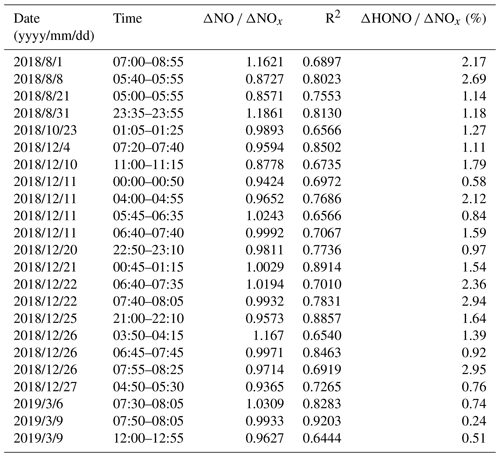## 3.3 Nighttime heterogeneous conversion of NO2 to HONO

### 3.3.1 Conversion rate of NO2 to HONO

Nighttime HONOcorr concentrations can be estimated from the heterogeneous conversion reaction (Meusel et al., 2016; Alicke, 2002; Su et al., 2008a). Although the mechanism of the nighttime HONO heterogeneous reaction is unclear, the formula for the heterogeneous conversion (${C}_{\mathrm{HONO}}^{\mathrm{0}}$) of NO2 to HONO can be expressed as

$\begin{array}{}\text{(3)}& {C}_{\mathrm{HONO}}^{\mathrm{0}}=\frac{{\left[{\mathrm{HONO}}_{\mathrm{corr}}\right]}_{{t}_{\mathrm{2}}}-{\left[{\mathrm{HONO}}_{\mathrm{corr}}\right]}_{{t}_{\mathrm{1}}}}{\left({t}_{\mathrm{2}}-{t}_{\mathrm{1}}\right)×\stackrel{\mathrm{‾}}{\left[{\mathrm{NO}}_{\mathrm{2}}\right]}},\end{array}$

where $\stackrel{\mathrm{‾}}{\left[{\mathrm{NO}}_{\mathrm{2}}\right]}$ is the mean value of NO2 concentration between t1 and t2. Equation (4) has been suggested as a way to avoid the interference of direct emissions and diffusion (Su et al., 2008a):

$\begin{array}{}\text{(4)}& \begin{array}{rl}{C}_{\mathrm{HONO}}^{X}& =\frac{\left(\frac{\left[{\mathrm{HONO}}_{\mathrm{corr}}{\right]}_{\left({t}_{\mathrm{2}}\right)}}{{\left[X\right]}_{{t}_{\mathrm{2}}}}-\frac{\left[{\mathrm{HONO}}_{\mathrm{corr}}{\right]}_{\left({t}_{\mathrm{1}}\right)}}{\left[X{\right]}_{\left({t}_{\mathrm{1}}\right)}}\right)\stackrel{\mathrm{‾}}{\left[X\right]}}{\left({t}_{\mathrm{2}}-{t}_{\mathrm{1}}\right)\frac{\mathrm{1}}{\mathrm{2}}\left(\frac{\left[{\mathrm{NO}}_{\mathrm{2}}{\right]}_{\left({t}_{\mathrm{2}}\right)}}{\left[X{\right]}_{\left({t}_{\mathrm{2}}\right)}}+\frac{\left[{\mathrm{NO}}_{\mathrm{2}}{\right]}_{\left({t}_{\mathrm{1}}\right)}}{{\left[X\right]}_{\left({t}_{\mathrm{1}}\right)}}\right)\stackrel{\mathrm{‾}}{\left[X\right]}}\\ & =\frac{\mathrm{2}\left(\frac{\left[{\mathrm{HONO}}_{\mathrm{corr}}{\right]}_{\left({t}_{\mathrm{2}}\right)}}{{\left[X\right]}_{{t}_{\mathrm{2}}}}-\frac{\left[{\mathrm{HONO}}_{\mathrm{corr}}{\right]}_{\left({t}_{\mathrm{1}}\right)}}{\left[X{\right]}_{\left({t}_{\mathrm{1}}\right)}}\right)}{\left({t}_{\mathrm{2}}-{t}_{\mathrm{1}}\right)\left(\frac{\left[{\mathrm{NO}}_{\mathrm{2}}{\right]}_{\left({t}_{\mathrm{2}}\right)}}{\left[X{\right]}_{\left({t}_{\mathrm{2}}\right)}}+\frac{\left[{\mathrm{NO}}_{\mathrm{2}}{\right]}_{\left({t}_{\mathrm{1}}\right)}}{{\left[X\right]}_{\left({t}_{\mathrm{1}}\right)}}\right)},\end{array}\end{array}$

where [HONOcorr]t, [NO2]t, and [X]t were the concentrations of HONO, NO2, and species used for normalization (including NO2, CO, and black carbon in this study), respectively, at time t; $\stackrel{\mathrm{‾}}{X}$ is the average concentration of reference species between t1 and t2; and ${C}_{\mathrm{HONO}}^{X}$ represents the conversion rate normalized against reference species X (Su et al., 2008a). There were 86 cases meeting the criteria. Such a large number of cases contributes to the statistical analysis of the heterogeneity of HONO formation. The average values of ${C}_{\mathrm{HONO}}^{\mathrm{0}}$, ${C}_{\mathrm{HONO}}^{{\mathrm{NO}}_{\mathrm{2}}}$, ${C}_{\mathrm{HONO}}^{\mathrm{CO}}$, and ${C}_{\mathrm{HONO}}^{\mathrm{BC}}$ were 0.48 % h−1, 0.46 % h−1, 0.46 % h−1, and 0.46 % h−1, respectively. The combined ${C}_{\mathrm{HONO}}^{\mathrm{C}}$ was 0.46 % h−1. The average CHONO values obtained using different normalization methods agreed well. Therefore, an estimation value of 0.46 % h−1 should be suitable for the nighttime conversion rate from NO2 to HONO.

We also compared the conversion rates calculated in this study with other experiments. As shown in Table 3, ${C}_{\mathrm{HONO}}^{\mathrm{C}}$ varied widely, from 0.29 % h−1 to 2.40 % h−1, which may be due to the various kinds of land surface in the various environments. The ${C}_{\mathrm{HONO}}^{\mathrm{C}}$ in Xiamen is comparable to those derived in Shanghai (0.70 % h−1; Wang et al., 2013), Jinan (0.68 % h−1, D. Li et al., 2018), and Hong Kong (0.52 % h−1, Xu et al., 2015), less than the values calculated from most other sites, including Xinken (1.60 % h−1, Su et al., 2008a)), Guangzhou (2.40, Li et al., 2012), Spain (1.50, Sörgel et al., 2011), Beijing (0.80; Wang et al., 2017), the eastern Bohai Sea (1.80 % h−1, Wen et al., 2019), and Kathmandu (1.40 % h−1, Yu et al., 2009), but more than the value obtained in Shandong (0.29 % h−1, Wang et al., 2015). The highest ${C}_{\mathrm{HONO}}^{\mathrm{C}}$ was found in summer, with a value of 0.55 % h−1, which will be explained in Sect. 3.3.2. Another study also found that the highest ${C}_{\mathrm{HONO}}^{\mathrm{C}}$ (1.00 % h−1) appeared in summer (Wang et al., 2017).

Table 3Overview of the conversion frequencies from NO2 to HONO in Xiamen and comparisons with other studies.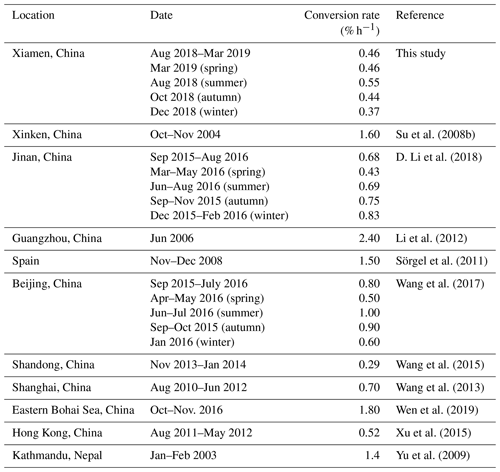### 3.3.2 The influence factors on HONO formation

The hydrolysis of NO2 on wet surfaces producing HONO is first-order affected by the concentration of NO2 (Finlayson-Pitts et al., 2003; Jenkin et al., 1988) and the absorption of water on the surfaces (Finlayson-Pitts et al., 2003; Kleffmann et al., 1998). A scatter plot of HONOcorr$/$ NO2 vs. RH is shown in Fig. 5. We calculated the top-five HONOcorr$/$ NO2 ratios in every 5 % RH interval based on a method introduced in previous literature (Li et al., 2012; Stutz et al., 2004), which will reduce the influence of those circumstances such as advection, the time of the night, and the surface density. These averaged maxima and standard deviations are shown in Fig. 5 as orange squares, except where data were sparse in a particular 5 % RH interval.

As for autumn and winter, the influence of RH on HONOcorr$/$ NO2 can be divided into two parts. The RH promoted an increase in HONOcorr$/$ NO2 for RH values less than 77.96 % in autumn and 91.99 % in winter, which is in line with the reaction kinetics of Reaction (R5). However, RH inhibits the conversion of NO2 to HONO when RH is higher than a turning point. According to many previous studies, water droplets will be formed on the surface of the ground or of aerosols when RH exceeds a certain value, thus resulting in a negative dependence of HONOcorr$/$ NO2 on RH (He et al., 2006; Zhou et al., 2007). A similar phenomenon was also found in Guangzhou and in Shanghai (70 %, Li et al., 2012; Wang et al., 2013) and in Kathmandu and in Beijing (65 %, Yu et al., 2009; Wang et al., 2017). However, in summer, RH appeared to promote the increase in HONOcorr$/$ NO2 without a turning point, suggesting that HONO production at night in summer strongly depends on RH. Another study also found a similar phenomenon in the summer in Guangzhou (Qin et al., 2009). This phenomenon might be caused by water droplets being evaporated by high temperatures. This is the reason for the highest ${C}_{\mathrm{HONO}}^{\mathrm{C}}$ in summer. As for spring, the relationship between HONOcorr$/$ NO2 and RH is very complicated and needs to be explored further in the future.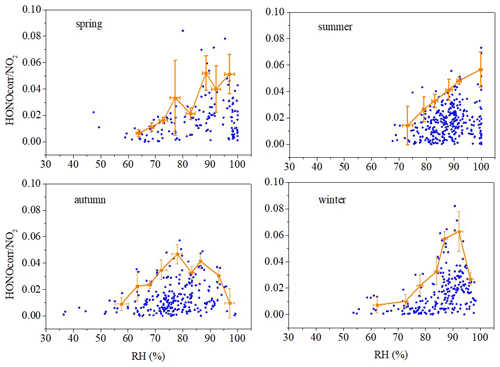Figure 5Scatter plots of nighttime HONOcorr$/$ NO2 ratios versus RH. The average top-five HONOcorr$/$ NO2 in every 5 % RH interval are shown as orange squares, and the error bars show ±1 SD.

It has been found that NH3 promoted hydrolysis of NO2 and production of HONO and NH4NO3 (Xu et al., 2019; L. Li et al., 2018). The correlations between the HONOcorr$/$ NO2 ratio, the NO${}_{\mathrm{3}}^{-}$$/$ NO2 ratio, and the NH3 concentration in four seasons were examined to investigate the influence of NH3 on HONO formation through promoting hydrolysis of NO2. Only nighttime data with RH above 80 % were chosen to avoid daytime rapid photolysis of HONO and enough water for NO2 quick hydrolysis (Xu et al., 2019). As shown in Fig. 6, for summer, the correlations between NH3 and the HONOcorr$/$ NO2 ratio was very poor and even negative ($R=-\mathrm{0.0438}$), and the correlation between the NO${}_{\mathrm{3}}^{-}$$/$ NO2 ratio and NH3 was also negative (0.2908). These results indicated that NH3 played a minor role in HONO production in summer. For autumn, although the NO${}_{\mathrm{3}}^{-}$$/$ NO2 ratio correlated well with NH3 (R= 0.3965) in autumn, the HONOcorr$/$ NO2 ratio had a negative correlation with NH3 ($R=-\mathrm{0.1305}$), which also indicated that NH3 played a minor role in HONO production in autumn. For spring, the correlation coefficient between the HONOcorr$/$ NO2 ratio and the NH3 concentration was the highest among the four seasons (0.3662), and the correlation between the NO${}_{\mathrm{3}}^{-}$$/$ NO2 ratio and the NH3 concentration was positive (0.1716). These phenomena proved that NH3 might promote HONO and NH4NO3 production through promoting NO2 hydrolysis in spring. For winter, positive correlations were found between NH3 and both the HONO $/$ NO2 ratio (R=0.1718) and NO${}_{\mathrm{3}}^{-}$$/$ NO2 ratio (R=0.2543), which indicated that NH3 might promote NO2 hydrolysis and HONO production in winter. All in all, NH3 might promote NO2 hydrolysis and HONO production in spring and winter, whereas NH3 played a minor role in HONO production in summer and autumn.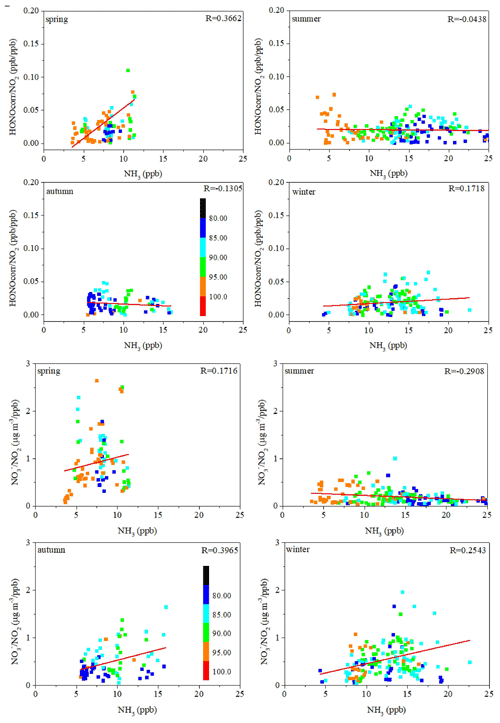Figure 6The correlation between the NH3 concentration and HONO $/$ NO2 ratio (upper) and the correlation between the NH3 concentration and NO${}_{\mathrm{3}}^{-}$$/$ NO2 (lower) in four seasons. The scatter points were colored by ambient RH values.

As shown in Fig. S3, HONOcorr$/$ NO2 reached a pseudo-steady state from 03:00 to 06:00 LT every night. A correlation analysis of HONOcorr$/$ NO2 with PM2.5 was carried out in the pseudo-steady state to understand the impact of aerosols on HONO production. Although we did not measure the aerosol surface density, the aerosol mass concentration can be used to replace this parameter (Huang et al., 2017; Park et al., 2004; Cui et al., 2018). The positive correlation of HONOcorr with PM2.5 (R1=0.4987) (Fig. 7a) may be a result of atmospheric physical processes such as convergence and diffusion. Using the HONOcorr$/$ NO2 ratio instead of a single HONO concentration for correlation analysis with PM2.5 reduced the impact of physical processes and indicated the extent of conversion of NO2 to HONO. Therefore, it was more credible that HONOcorr$/$ NO2 would be moderately positively correlated with PM2.5 (R2= 0.2331) during the whole observation period (Fig. 7b). As denoted by larger green squares in the Fig. 7b, HONOcorr$/$ NO2 correlated well with PM2.5 when its concentration was higher than 35 µg m−3 (R3= 0.4568). The larger the amount of HONO produced by the heterogeneous reaction of NO2 on the aerosol surface, the better the correlation between HONO $/$ NO2 and PM2.5 (Cui et al., 2018; Wang et al., 2003; Hou et al., 2016; Li et al., 2012; Nie et al., 2015).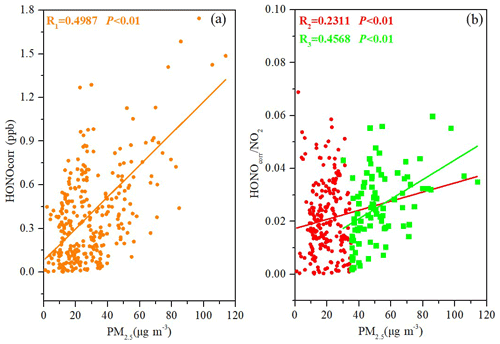Figure 7The correlation between PM2.5 and HONOcorr (a) and the correlation between PM2.5 and HONOcorr$/$ NO2 (b). The squares depict PM2.5 35 µg m−3; all scattered points are from the time when the ratio of HONOcorr$/$ NO2 reached a pseudo-steady state each night (03:00–06:00 LT).

## 3.4 Daytime sources of HONO

### 3.4.1 Budget analysis of HONO

Having discussed the nighttime chemical behavior of HONO, we now concentrate on the daytime chemical behavior of HONO. Here, Runknown is used to stand for the rate of emission from unknown sources. The value of Runknown was estimated based on the balance between sources and sinks due to its short atmospheric lifetime. The sources are (1) oxidation of NO by OH (${R}_{\mathrm{OH}+\mathrm{NO}}={k}_{\mathrm{OH}+\mathrm{NO}}\left[\mathrm{NO}\right]\left[\mathrm{OH}\right]$), (2) dark heterogeneous production (Phet), and (3) direct vehicle emission (Pemis); the sinks are (1) HONO photolysis (Rphot=JHONO[HONO]), (2) oxidation of HONO by OH (${R}_{\mathrm{OH}+\mathrm{HONO}}={k}_{\mathrm{OH}+\mathrm{HONO}}\left[\mathrm{HONO}\right]\left[\mathrm{OH}\right]$), and (3) dry deposition (Ldep). The value of Runknown can then be calculated according to

$\begin{array}{}\text{(5)}& \begin{array}{rl}{R}_{\mathrm{unknown}}& ={J}_{\mathrm{HONO}}\left[\mathrm{HONO}\right]+{k}_{\mathrm{OH}+\mathrm{HONO}}\left[\mathrm{HONO}\right]\left[\mathrm{OH}\right]\\ & +{L}_{\mathrm{dep}}+\frac{\mathrm{\Delta }\left[\mathrm{HONO}\right]}{\mathrm{\Delta }t}-{k}_{\mathrm{OH}+\mathrm{NO}}\left[\mathrm{NO}\right]\left[\mathrm{OH}\right]\\ & -{P}_{\mathrm{het}}-{P}_{\mathrm{emis}},\end{array}\end{array}$

where ${k}_{\mathrm{OH}+\mathrm{HONO}}=$ 6.0 × 10−12 cm3 molecules−1 s−1 and ${k}_{\mathrm{OH}+\mathrm{NO}}=$ 7.4 × 10−12 cm3 molecules−1 s−1, values cited from a previous study (Sörgel et al., 2011). The OH concentration ([OH]) was estimated in this study because no data for this value were available. An improved empirical formula, Eq. (6), was applied to estimate [OH] using the NO2 and HONO concentrations and the photolysis rate constants (J) of NO2, O3, and HONO (Wen et al., 2019). Equation (6) fully considers the influence of photolysis and precursors on the concentration of [OH].

$\begin{array}{}\text{(6)}& \begin{array}{rl}\left[\mathrm{OH}\right]& =\mathrm{4.1}×{\mathrm{10}}^{\mathrm{9}}\\ & ×\frac{\begin{array}{c}J\left({\mathrm{O}}^{\mathrm{1}}\mathrm{D}{\right)}^{\mathrm{0.83}}×J\left({\mathrm{NO}}_{\mathrm{2}}{\right)}^{\mathrm{0.19}}×\left(\mathrm{140}×{\mathrm{NO}}_{\mathrm{2}}+\mathrm{1}\right)\\ +\mathrm{HONO}×J\left(\mathrm{HONO}\right)\end{array}}{\begin{array}{c}\mathrm{0.41}×{\mathrm{NO}}_{\mathrm{2}}^{\mathrm{2}}+\mathrm{1.7}×{\mathrm{NO}}_{\mathrm{2}}+\mathrm{1}+\mathrm{NO}×{k}_{\mathrm{NO}+\mathrm{OH}}\\ +\mathrm{HONO}×{k}_{\mathrm{HONO}+\mathrm{OH}}\end{array}}\end{array}\end{array}$

During spring, summer, autumn, and winter, the average midday OH concentrations were 8.86 × 106, 1.48 × 107, 1.36 × 107, and 6.19 × 106 cm−3, respectively, which were within the range of those obtained in other studies varying from 4 × 106 to 1.7 × 107 cm−3 (Tan et al., 2017; Lu et al., 2013).

$\frac{\mathrm{\Delta }\left[\mathrm{HONO}\right]}{\mathrm{\Delta }t}$ is the observed change of HONO concentration (ppb s−1). The value of $\frac{\mathrm{\Delta }\left[\mathrm{HONO}\right]}{\mathrm{\Delta }t}$ is the concentration difference between the center of one interval (1 min) and the center of the next interval, and this accounts for changes in concentration levels (Sörgel et al., 2011). The parameter Ldep can be quantified by multiplying the dry deposition rate of HONO by the observed HONO concentration and then dividing by the mixing layer height $\left({L}_{\mathrm{dep}}=\frac{{\mathit{\nu }}_{\mathrm{HONO}}^{\mathrm{ground}}×\left[\mathrm{HONO}\right]}{H}\right)$. A value of ${\mathit{\nu }}_{\mathrm{HONO}}^{\mathrm{ground}}=$ 2 cm s−1 was used for the deposition rate (Sörgel et al., 2011; Su et al., 2008a). Although the mixing layer heights during spring, summer, autumn, and winter were 1074.4, 1173.8, 1494.6, and 1310.4 m, respectively (Gao, 1999), most HONO cannot reach the height of 200 m due to rapid photolysis of HONO during the daytime. Therefore, the mixing layer height 200 m was used to parameterize Ldep. In summarizing the known HONO sources, we included the nighttime heterogeneous production as a known source based on the assumption that the day continues in the same way as the night (Sörgel et al., 2011). The term Phete was parameterized by NO2 conversion at night using the formula ${P}_{\mathrm{hete}}={C}_{\mathrm{HONO}}^{\mathrm{C}}\left[{\mathrm{NO}}_{\mathrm{2}}\right]$ (Alicke, 2002).

Figure 8 shows the contributions of each term in Eq. (7) to the HONO budgets in different seasons. Photolysis of HONO (Rphot) formed the largest proportion of the sinks in all four seasons, accounting for 87.85 %, 88.79 %, 88.15 %, and 86.71 % in spring, summer, autumn, and winter, respectively. The value of Rphot in summer was the highest (3.60 ppb h−1), followed by spring (3.08 ppb h−1), autumn (2.38 ppb h−1), and winter (2.26 ppb h−1). The oxidation of HONO by OH contributed little to HONO sinks (2.77 % of all sinks). Dry deposition (Ldep) was also very small (9.35 % of all sinks). As for known sources, ROH+NO was the main known source in all four seasons, wherein the largest proportion was found in summer (64.44 %), followed by autumn (53.66 %), spring (53.25 %), and winter (51.73 %). Direct emission was second among the known sources, accounting for 38.36 %, 27.49 %, 37.02 %, and 40.81 % in spring, summer, autumn, and winter, respectively. Dark heterogeneous formation (Phete) was almost negligible in the daytime, accounting for approximately 8.31 % of known sources during the whole observation period. As for unknown sources, these made up the largest proportion of all sources found in summer (81.25 %), followed by autumn (73.99 %), spring (70.87 %) and winter (59.28 %).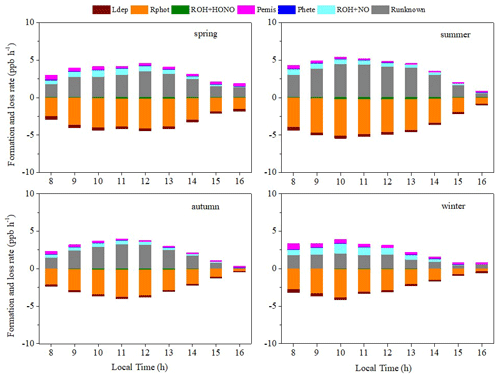Figure 8Average diurnal variations of each source (> 0) and sink (< 0) of HONO in the four seasons.

It is worth noting that Runknown exhibited a maximum around noon in all seasons. A previous study in Wangdu (Liu et al., 2019b) also found that unknown sources of HONO reached a maximum at midday, with the strongest photolysis rates in summer. This strengthens the validity of the assumption that the missing HONO formation mechanism is related to a photolytic source (Michoud et al., 2014). In the present study, the daily maximum Runknown value was 4.51 ppb h−1 in summer, followed by 3.51 ppb h−1 in spring, 3.28 ppb h−1 in autumn, and 2.08 ppb h−1 in winter. Average Runknown during the whole observation was 2.32 ppb h−1, which was almost at the upper–middle level of studies reported: 0.5 ppb h−1 in a forest near Jülich, Germany (Kleffmann, 2005); 0.77 ppb h−1 at a rural site in the Pearl River delta, China (Li et al., 2012); 1.04 ppb h−1 at a suburban site in Nanjing, China (Liu et al., 2019a);  2 ppb h−1 in Xinken, China (Su et al., 2008a); and 2.95 ppb h−1 in the urban atmosphere of Jinan, China (D. Li et al., 2018).

### 3.4.2 Exploration of possible unknown daytime sources

According to the analyses in Sects. 3.1 and 3.4.1, the unknown sources are likely to be related to light. It was indeed found that the unknown sources have a good correlation with the parameters related to light. It was reported in previous studies that particulate nitrate photolysis is a source of HONO (Ye et al., 2017, 2016; Scharko et al., 2014; Romer et al., 2018; McFall et al., 2018). We will discuss the possibility of HONO being produced by photolysis of particulate nitrate (J(NO3_R) ×pNO${}_{\mathrm{3}}^{-}$) at this site in this section. There was a logarithmic relationship showing good correlation between Runknown (ppb h−1) and J(NO${}_{\mathrm{3}}^{-}$_R) ×pNO${}_{\mathrm{3}}^{-}$ (µg m−3 s−1) in spring (R2= 0.6519) and summer (R2= 0.6511), while relatively weak correlation was found in autumn (R2= 0.3633) and winter (R2= 0.4186) (Fig. 9). This result indicated that photolysis of particulate nitrate contributed more in spring and summer than in autumn and winter. In conditions of relatively lower J(NO3_R) ×pNO${}_{\mathrm{3}}^{-}$, Runknown increased rapidly with increasing pNO${}_{\mathrm{3}}^{-}$ concentration and its photolysis rate constant but reached a plateau after a critical value (J(NO3_R) ×pNO${}_{\mathrm{3}}^{-}$>  0.5 µg m−3 s−1 in summer, J(NO3_R) ×pNO${}_{\mathrm{3}}^{-}$> 0.4 µg m−3 s−1 in autumn, and J(NO3_R) ×pNO${}_{\mathrm{3}}^{-}$> 1.5 µg m−3 s−1 in winter). There was no obvious turning point in spring, but it could be seen that the growth rate was declining. This indicated that in conditions that were relatively cleaner, the missing daytime source of HONO was limited by the pNO${}_{\mathrm{3}}^{-}$ concentration and the photolysis rate constant. However, with enough particulate nitrate providing sufficient precursor or enough light to stimulate the reaction, the HONO production did not increase as J(NO3_R) ×pNO${}_{\mathrm{3}}^{-}$ increased. Other generation mechanisms might play leading roles in the condition with enough particulate nitrate or enough light. It was found in a previous study that heterogeneous soot photochemistry may contribute to the daytime HONO concentration (Monge et al., 2010). Black carbon (BC) values were used as a substitute for soot values (Sörgel et al., 2011). When BC concentration was above 2.0 µg m−3, the missing daytime source of HONO did not increase as J(NO3_R) ×pNO${}_{\mathrm{3}}^{-}$ increased. We found that the missing daytime source of HONO correlated better with BC × UV (R= 0.9269, R= 0.6356) than with BC (R= 0.4776, R= 0.6071) or UV (R= 0.8494, R= 0.4262) alone in autumn and winter (Fig. S4), probably related to the conversion of NO2 to HONO on BC enhanced by light.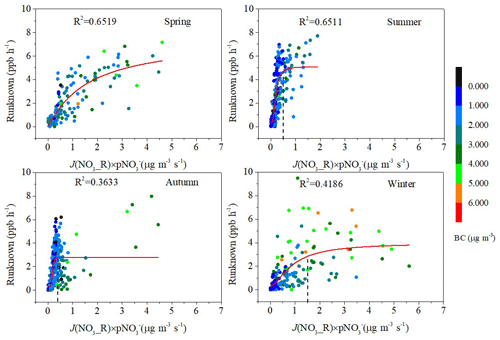Figure 9Relationships between the photolysis of particulate nitrate and Runknown, colored by BC in spring, summer, autumn, and winter. Red lines and dashed lines represent logarithmic fitting curve and turning point, respectively.

We discuss whether photolysis of particulate nitrate was able to provide enough additional HONO by estimating the rate of HONO production by nitrate photolysis in spring and summer (Zhou et al., 2007; Li et al., 2012; Wang et al., 2017) using

$\begin{array}{}\text{(7)}& {J}_{{\mathrm{NO}}_{\mathrm{3}}^{-}\to \mathrm{HONO}}=\frac{{R}_{\mathrm{unknown}}×H}{f×\left[{\mathrm{NO}}_{\mathrm{3}}^{-}\right]×{\mathit{\upsilon }}_{{\mathrm{NO}}_{\mathrm{3}}^{-}}×{t}_{\mathrm{d}}},\end{array}$

where ${J}_{{\mathrm{NO}}_{\mathrm{3}}^{-}\to \mathrm{HONO}}$ is the rate of photolysis of ${\mathrm{NO}}_{\mathrm{3}}^{-}$ to form HONO, ${\mathit{\upsilon }}_{{\mathrm{NO}}_{\mathrm{3}}^{-}}$ is the dry deposition rate of ${\mathrm{NO}}_{\mathrm{3}}^{-}$ during the period td, and f is the proportion of the surface exposed to the sun at midday. Here, we suppose that the surfaces involving ${\mathrm{NO}}_{\mathrm{3}}^{-}$ were exposed to light by a factor $f=\mathrm{1}/\mathrm{4}$, taking mixing height H=200 m and ${\mathit{\upsilon }}_{{\mathrm{NO}}_{\mathrm{3}}^{-}}=$ 5 cm s−1 over td= 24 h. We use the mean midday value of Runknown= 9.72 µg m−3 h−1 and $\left[{\mathrm{NO}}_{\mathrm{3}}^{-}\right]=$ 10.35 µg m−3 in spring and Runknown= 11.51 µg m−3 h−1 and $\left[{\mathrm{NO}}_{\mathrm{3}}^{-}\right]=$ 2.86 µg m−3 in summer. The photolysis rates ${J}_{{\mathrm{NO}}_{\mathrm{3}}^{-}\to \mathrm{HONO}}$ derived from Eq. (8) were 4.83 × 10−5 s−1 and 2.07 × 10−4 s−1 for spring and summer, respectively. These values were in the range 6.2 × 10−6 to 5.0 × 10−4 obtained in a previous study (Ye et al., 2017), which indicated that particulate nitrate photolysis could be a likely source for the missing daytime additional HONO formation in spring and summer. The variability of ${J}_{{\mathrm{NO}}_{\mathrm{3}}^{-}\to \mathrm{HONO}}$ may be caused by chemical composition, acidity, light-absorbing constituents, and the optical and other physical properties of aerosols.

## 3.5 Parameterization of HONO

Through an empirical parameterized formula, we can explore an accurate parameterization method for HONO, discuss the main control factors for the HONO concentration and its chemical behavior, and quantify its main sources and key kinetic parameters. As mentioned in Sect. 3.1, the HONO $/$ NOx ratio is better than HONO $/$ NO2 as an indicator of HONO generation. In another study (Elshorbany et al., 2012), data were collected from 15 field observations all over the world to establish the correlation between the HONO $/$ NOx ratio and the HONO concentration in global models. Therefore, we applied this method in this study to parameterize the HONO concentration. As shown in Fig. 10, the ΔHONO $/$ΔNOx ratios in the four seasons were close to the calculated value (0.02). However, there were seasonal variations in the slope, showing a maximum in summer (2.60 × 10−2), followed by autumn (2.06 × 10−2), and a minimum in winter (1.59 × 10−2). Except for in spring, HONO showed good correlation with NOx, with R2 values ranging from 0.8972 to 0.9621. Therefore, we used slopes of 2.60 × 10−2, 2.06 × 10−2, and 1.59 × 10−2 to parameterize the HONO concentrations in summer, autumn, and winter, respectively. As for spring, though only a weak correlation between HONO and NOx was found, the majority of the ΔHONO $/$ΔNOx ratios fluctuated round a slope of 0.02 because concentrations of NOx greater than 60 ppb only accounted for 8.83 % of the data. Therefore, a slope of 0.02 was applied in spring to parameterize the HONO concentration.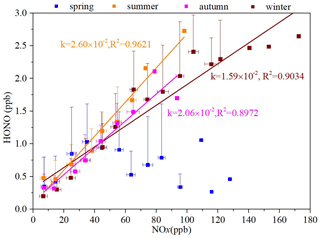Figure 10The ratio of HONO $/$ NOx in the four seasons (correlation between the average of NOx per 10 ppb interval and the average value of HONO).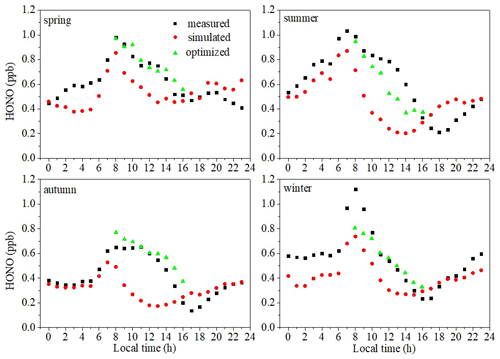Figure 11The diurnal variations in the measured values of HONO (black squares), the estimated values of HONO using the parameterized formula (red circles), and the estimated values of HONO using the parameterized formula combined with the main daytime sources (green triangles).

As can be seen from Fig. 11, the estimated values are very close to the observed values in the nighttime in autumn. After sunrise and before noon, the values observed were higher than the estimated values, and this difference gradually increases. After noon and before sunset, the values observed were still higher than the values estimated, but the difference gradually decreases. This phenomenon was also found in the daytime in spring and summer, but not in winter. Compared with the daytime, the estimated values during the nighttime were closer to the observed values in both trend and value in all four seasons, which further demonstrates that nighttime HONO is mainly produced from the direct vehicle emissions and heterogeneous reaction of NO2 on the ground or the surfaces of aerosols. Therefore, we should pay much more attention to simulation in the daytime. We distinguish two main sectors, nighttime and daytime, to analyze the factors affecting the HONO diurnal variation (Liu, 2017). Although J(HONO HONO also correlated well with J(NO2) × NO2 in all four seasons in this study and the linear fitting coefficients fluctuated around 0.01 in all four seasons (Fig. S5), bad simulation results during the daytime were found (Fig. S6) using

$\begin{array}{}\text{(8)}& \left[\mathrm{HONO}\right]=k×\left[{\mathrm{NO}}_{\mathrm{2}}\right]×J\left({\mathrm{NO}}_{\mathrm{2}}\right)/J\left(\mathrm{HONO}\right),\end{array}$

where k was the linear fitting coefficient between J(HONO HONO and J(NO2) × NO2. In contrast, excellent simulation results were found in a previous study using the same formula (Liu, 2017), which suggests that using the same simulation formula in different regions may obtain greatly varying results. Equation (8) can be regarded as a combination of [NO2] with J(NO2) $/$J(HONO). J(NO2) $/$J(HONO) stayed relatively constant (5.48–5.87) in the daytime in four seasons. Therefore, diurnal variation of [HONO] simulated by Eq. (8) depended on [NO2] (Fig. S7). Equation (8) is only suitable for regions where the diurnal variation of [NO2] is consistent with that of [HONO].

As discussed in Sect. 3.4.2, nitrate photolysis was perhaps the source of HONO in this study. Besides, the difference between the observed value and the simulated value kept increasing before noon, and the difference began to decrease after noon, which was consistent with nitrate photolysis. Therefore, we take the photolysis of nitrate into the HONO concentration simulation. The specific formulas for the simulation of spring, summer, autumn, and winter are shown as follows.

$\begin{array}{}\text{(9)}& \begin{array}{rl}{\mathrm{HONO}}_{\mathrm{spring}}& =\mathrm{2.00}×{\mathrm{10}}^{-\mathrm{2}}×{\mathrm{NO}}_{x}+\left[{\mathrm{NO}}_{\mathrm{3}}^{-}\right]\\ & ×J\left({\mathrm{NO}}_{\mathrm{3}}\mathit{_}\mathrm{R}\right)/\mathrm{4}\end{array}\text{(10)}& \begin{array}{rl}{\mathrm{HONO}}_{\mathrm{summer}}& =\mathrm{2.60}×{\mathrm{10}}^{-\mathrm{2}}×{\mathrm{NO}}_{x}+\left[{\mathrm{NO}}_{\mathrm{3}}^{-}\right]\\ & ×J\left({\mathrm{NO}}_{\mathrm{3}}\mathit{_}\mathrm{R}\right)\end{array}\text{(11)}& \begin{array}{rl}{\mathrm{HONO}}_{\mathrm{autumn}}& =\mathrm{2.06}×{\mathrm{10}}^{-\mathrm{2}}×{\mathrm{NO}}_{x}+\left[{\mathrm{NO}}_{\mathrm{3}}^{-}\right]\\ & ×J\left({\mathrm{NO}}_{\mathrm{3}}\mathit{_}\mathrm{R}\right)\end{array}\text{(12)}& \begin{array}{rl}{\mathrm{HONO}}_{\mathrm{winter}}& =\mathrm{1.59}×{\mathrm{10}}^{-\mathrm{2}}×{\mathrm{NO}}_{x}+\left[{\mathrm{NO}}_{\mathrm{3}}^{-}\right]\\ & ×J\left({\mathrm{NO}}_{\mathrm{3}}\mathit{_}\mathrm{R}\right)/\mathrm{4}\end{array}\end{array}$

In this way, the daytime simulation results are significantly improved (Fig. 11). This further demonstrates that the apportionment of HONO sources is credible. The parameterization described in this work was more reasonable and can be better used in the future in such coastal sites.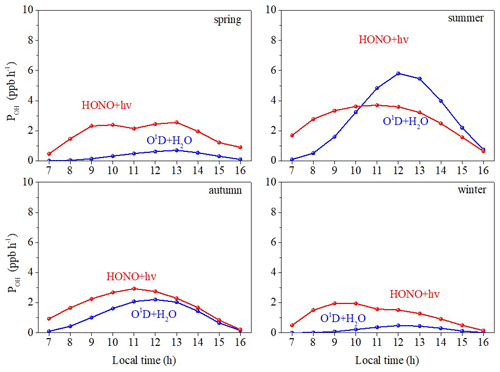Figure 12Comparison of OH formation by photolysis of HONO and O3 in the four seasons.

## 3.6 Comparison of contributions of HONO and O3 to OH radicals

Comparing the OH radical production via photolysis of HONO and O3, the effect of the high HONO concentrations in the daytime on the tropospheric oxidation capacity was evaluated (Ryan et al., 2018). Nitrous acid is considered to be a crucial source of OH radicals (Lee et al., 2016). As shown in Eq. (12), OH production rates from O3 photolysis (POH(O3)) were calculated based on [O3], J(O1D), and [H2O] (Liu et al., 2019a). Only O(1D) atoms produced by the O3 photolysis at UV wavelengths less than 320 nm (Reaction R6) can combine with water to generate OH radicals (Reaction R7) in the atmosphere. The absolute water concentration was derived from temperature and RH. The Reaction (R8) rate for N2 is 3.1 × 10−11 cm3 molecules−1 s−1 and for O2 is 4.0 × 10−11 cm3 molecules−1 s−1 (Liu et al., 2019a). The net OH formation from HONO was estimated by Eq. (13) (Su et al., 2008a; Sörgel et al., 2011; D. Li et al., 2018; Atkinson et al., 2004). In addition to the two primary production modes of OH radicals mentioned above, there are the reaction of organic and hydro-peroxy radicals (RO2 and HO2) with NO, hydrogen peroxide photolysis, and the ozonolysis of alkenes (Hofzumahaus et al., 2009; Gligorovski et al., 2015; Wang et al., 2018).

$\begin{array}{ll}& {P}_{\mathrm{OH}}\left({\mathrm{O}}_{\mathrm{3}}\right)=\mathrm{2}J\left({\mathrm{O}}^{\mathrm{1}}\mathrm{D}\right)\left[{\mathrm{O}}_{\mathrm{3}}\right]\mathit{\varphi }\mathrm{OH},\mathit{\varphi }\mathrm{OH}\\ \text{(12)}& & ={k}_{\mathrm{7}}\left[{\mathrm{H}}_{\mathrm{2}}\mathrm{O}\right]/\left({k}_{\mathrm{7}}\left[{\mathrm{H}}_{\mathrm{2}}\mathrm{O}\right]+{k}_{\mathrm{8}}\left[\mathrm{M}\right]\right)\text{(R6)}& & {\mathrm{O}}_{\mathrm{3}}+hv\to \mathrm{O}\left({}^{\mathrm{1}}\mathrm{D}\right)+{\mathrm{O}}_{\mathrm{2}}\left(hv\mathit{<}\phantom{\rule{0.125em}{0ex}}\mathrm{320}\phantom{\rule{0.125em}{0ex}}\mathrm{nm}\right)\text{(R7)}& & \mathrm{O}\left({}^{\mathrm{1}}\mathrm{D}\right)+{\mathrm{H}}_{\mathrm{2}}\mathrm{O}\to \mathrm{2}\mathrm{OH}\text{(R8)}& & \mathrm{O}\left({}^{\mathrm{1}}\mathrm{D}\right)+\mathrm{M}\to \mathrm{O}\left({}^{\mathrm{3}}P\right)+\mathrm{M}\left(\mathrm{M}\phantom{\rule{0.25em}{0ex}}\mathrm{is}\phantom{\rule{0.25em}{0ex}}{N}_{\mathrm{2}}\phantom{\rule{0.25em}{0ex}}\mathrm{or}\phantom{\rule{0.25em}{0ex}}{\mathrm{O}}_{\mathrm{2}}\right)& {P}_{\mathrm{OH}}\left(\mathrm{HONO}\right)={J}_{\mathrm{HONO}}\left[\mathrm{HONO}\right]-{k}_{\mathrm{OH}+\mathrm{NO}}\left[\mathrm{NO}\right]\left[\mathrm{OH}\right]\\ \text{(13)}& & -{k}_{\mathrm{OH}+\mathrm{HONO}}\left[\mathrm{HONO}\right]\left[\mathrm{OH}\right]\end{array}$

The diurnal patterns of P(OH) are shown in Fig. 12. The formation rates of OH from O3 photolysis peaked at midday at around 0.71, 5.80, 2.21, and 0.48 ppb h−1 for spring, summer, autumn, and winter, respectively. The variation of POH(O3) is consistent with J(O1D) (Fig. S8), peaking at midday and in summer on a diurnal and a seasonal timescale, respectively. For summer and autumn, POH(HONO) had a similar trend to POH(O3), peaking at around noon at the time of the highest J(HONO), but this was negligible at sunrise and sunset (Fig. S9). For spring and winter, however, POH(HONO) reached a maximum in the morning rush hour caused by the combined influences of high HONO concentration and high J(HONO). A similar result was also found in southwestern Spain from mid-November to mid-December 2008 (Sörgel et al., 2011).The HONO photolysis contributed significantly more OH than O3 photolysis during the whole daytime in spring, autumn, and winter. In summer, the HONO photolysis contributed to more OH in the early morning, and although the O3 photolysis produced more in the afternoon, HONO photolysis had a considerable effect on OH production. A similar result was also found in Nanjing in eastern China from November 2017 to November 2018 (Liu et al., 2019a). These results show that HONO contributes considerably to the atmospheric oxidizing capacity of the suburban atmosphere of Xiamen. Although HONO concentrations (average: 0.66 ppb) are much lower than O3 concentrations (average: 35.88 ppb) during 07:00–16:00 LT, daytime HONO photolysis forms significantly more OH than daytime photolysis of O3 in four seasons except for summer afternoons. Generally, the mean value of POH(HONO) from 07:00 to 16:00 LT was 1.89 ppb h−1, and the average POH(O3) was 1.14 ppb h−1. A similar result was found in Melbourne, where the peak OH production rate reached 2 ppb h−1 from 0.4 ppb HONO (Ryan et al., 2018). The important role of HONO in the production of OH promotes photochemical peroxyacetyl nitrate formation (Hu et al., 2020).

4 Conclusions

We conducted measurements of HONO in the atmosphere at an IUE supersite in a coastal city of southeastern China in August, October, and December 2018 and March 2019, finding an average HONO concentration of 0.54 ± 0.47 ppb across the whole observation period. Concentrations of HONO in spring and summer were higher than in winter and autumn, which was consistent with seasonal variations in RH. Both higher HONO concentrations in the daytime and the HONO $/$ NOx ratio peaking around noon suggested that additional sources of HONO might be related to light. It was found that the contribution from vehicle exhaust emissions (1.45 %) was higher than that found in most other studies due to the site being surrounded by several expressways with a large number of passing diesel vehicles. The average nocturnal conversion rate of NO2 to HONO was 0.46 % h−1, which was within the range 0.29–2.40 % h−1 found by other studies. The HONOcorr$/$ NO2 ratio increased with RH and the concentration of PM2.5 during the nighttime, which indicates that nocturnal heterogeneous reactions on the surfaces of aerosols are the major source of HONO. However, dark heterogeneous formation (Phete) was almost negligible in the daytime, accounting for approximately 8.31 % of known sources across the whole observation period. Runknown made up at the largest proportion of all sources in summer (81.25 %), autumn (73.99 %), spring (70.87 %), and winter (59.28 %). It was found that there was a logarithmic relationship between Runknown and particulate nitrate photolysis in four seasons. The variation of HONO at night can be accurately simulated based on the HONO $/$ NOx ratio, while J(NO${}_{\mathrm{3}}^{-}$_R) ×pNO${}_{\mathrm{3}}^{-}$ or $\mathrm{1}/\mathrm{4}×$ (J(NO${}_{\mathrm{3}}^{-}$_R) ×pNO${}_{\mathrm{3}}^{-}$) should be considered for daytime simulation. Local tropospheric oxidation capacity was significantly increased by HONO during 07:00–16:00 LT, providing an OH radical source (1.89 ppb h−1).

Data availability

The observation data at this site are available from the authors upon request.

Supplement

Author contributions

BH and JD contributed equally to this work. BH and JD collected the HONO data and analyzed the data. BH wrote the paper. BH and JD performed the experiments. JD and FW built the IBBCEEAS equipment. YH, MQ, and JC revised the paper. MQ, PX, and JC designed the paper. JC supported funding of observation and research. LX, ML, and YB contributed to discussions of results.

Competing interests

The contact author has declared that neither they nor their co-authors have any competing interests.

Disclaimer

Publisher's note: Copernicus Publications remains neutral with regard to jurisdictional claims in published maps and institutional affiliations.

Acknowledgements

This study was funded by the Cultivating Project of Strategic Priority Research Program of the Chinese Academy of Sciences (XDPB1903), the FJIRSM&IUE Joint Research Fund (RHZX-2019-006), the Center for Excellence in Regional Atmospheric Environment, CAS (E0L1B20201), State Key Laboratory of Environmental Chemistry and Ecotoxicology, Research Center for Eco-Environmental Sciences, CAS, and Xiamen Atmospheric Environment Observation and Research Station of Fujian Province.

Financial support

This research has been supported by the National Key Research and Development Program of China (grant nos. 2017YFC0209400, 2016YFC02005, and 2016YFC0112200) and the National Natural Science Foundation of China (grant nos. 41575146 and 41875154). This study was also funded by the Cultivating Project of Strategic Priority Research Program of the Chinese Academy of Sciences (XDPB1903), the FJIRSM&IUE Joint Research Fund (RHZX-2019-006), and the Center for Excellence in Regional Atmospheric Environment, CAS (E0L1B20201).

Review statement

This paper was edited by Steven Brown and reviewed by two anonymous referees.

References

Acker, K., Febo, A., Trick, S., Perrino, C., Bruno, P., Wiesen, P., Möller, D., Wieprecht, W., Auel, R., Giusto, M., Geyer, A., Platt, U., and Allegrini, I.: Nitrous acid in the urban area of Rome, Atmos. Environ., 40, 3123–3133, https://doi.org/10.1016/j.atmosenv.2006.01.028, 2006.

Alicke, B.: Impact of nitrous acid photolysis on the total hydroxyl radical budget during the Limitation of Oxidant Production/Pianura Padana Produzione di Ozono study in Milan, J. Geophys. Res., 107, 8196, https://doi.org/10.1029/2000jd000075, 2002.

Ammann, M., Kalberer, M., Jost, D. T., Tobler, L., Rössler, E., Piguet, D., Gäggeler, H. W., and Baltensperger, U.: Heterogeneous production of nitrous acid on soot in polluted air masses, Nature, 395, 157–160, 1998.

Atkinson, R., Baulch, D. L., Cox, R. A., Crowley, J. N., Hampson, R. F., Hynes, R. G., Jenkin, M. E., Rossi, M. J., and Troe, J.: Evaluated kinetic and photochemical data for atmospheric chemistry: Volume I – gas phase reactions of Ox, HOx, NOx and SOx species, Atmos. Chem. Phys., 4, 1461–1738, https://doi.org/10.5194/acp-4-1461-2004, 2004.

Aubin, D. G. and Abbatt, J. P.: Interaction of NO2 with hydrocarbon soot: Focus on HONO yield, surface modification, and mechanism, J. Phys. Chem. A, 111, 6263–6273, 2007.

Chang, Y., Zou, Z., Deng, C., Huang, K., Collett, J. L., Lin, J., and Zhuang, G.: The importance of vehicle emissions as a source of atmospheric ammonia in the megacity of Shanghai, Atmos. Chem. Phys., 16, 3577–3594, https://doi.org/10.5194/acp-16-3577-2016, 2016.

Cui, L., Li, R., Zhang, Y., Meng, Y., Fu, H., and Chen, J.: An observational study of nitrous acid (HONO) in Shanghai, China: The aerosol impact on HONO formation during the haze episodes, Sci. Total. Environ., 630, 1057–1070, https://doi.org/10.1016/j.scitotenv.2018.02.063, 2018.

Duan, J., Qin, M., Ouyang, B., Fang, W., Li, X., Lu, K., Tang, K., Liang, S., Meng, F., Hu, Z., Xie, P., Liu, W., and Häsler, R.: Development of an incoherent broadband cavity-enhanced absorption spectrometer for in situ measurements of HONO and NO2, Atmos. Meas. Tech., 11, 4531–4543, https://doi.org/10.5194/amt-11-4531-2018, 2018.

Elshorbany, Y. F., Kurtenbach, R., Wiesen, P., Lissi, E., Rubio, M., Villena, G., Gramsch, E., Rickard, A. R., Pilling, M. J., and Kleffmann, J.: Oxidation capacity of the city air of Santiago, Chile, Atmos. Chem. Phys., 9, 2257–2273, https://doi.org/10.5194/acp-9-2257-2009, 2009.

Elshorbany, Y. F., Steil, B., Brühl, C., and Lelieveld, J.: Impact of HONO on global atmospheric chemistry calculated with an empirical parameterization in the EMAC model, Atmos. Chem. Phys., 12, 9977–10000, https://doi.org/10.5194/acp-12-9977-2012, 2012.

Finlayson-Pitts, B. J., Wingen, L. M., Sumner, A. L., Syomin, D., and Ramazan, K. A.: The heterogeneous hydrolysis of NO2 in laboratory systems and in outdoor and indoor atmospheres: An integrated mechanism, Phys. Chem. Chem. Phys., 5, 223–242, https://doi.org/10.1039/b208564j, 2003.

Fu, X., Wang, T., Zhang, L., Li, Q., Wang, Z., Xia, M., Yun, H., Wang, W., Yu, C., Yue, D., Zhou, Y., Zheng, J., and Han, R.: The significant contribution of HONO to secondary pollutants during a severe winter pollution event in southern China, Atmos. Chem. Phys., 19, 1–14, https://doi.org/10.5194/acp-19-1-2019, 2019.

Gao, J.: An analysis of some pollution weather conditions, Journal of Oceanography in Taiwan Strait, 18, 55–62, 1999.

Ge, S., Wang, G., Zhang, S., Li, D., Xie, Y., Wu, C., Yuan, Q., Chen, J., and Zhang, H.: Abundant NH3 in China Enhances Atmospheric HONO Production by Promoting the Heterogeneous Reaction of SO2 with NO2, Environ. Sci. Technol., 53, 14339–14347, https://doi.org/10.1021/acs.est.9b04196, 2019.

George, C., Strekowski, R. S., Kleffmann, J., Stemmler, K., and Ammann, M.: Photoenhanced uptake of gaseous NO2 on solid organic compounds: a photochemical source of HONO?, Faraday Discuss., 130, 195–210, https://doi.org/10.1039/b417888m, 2005.

Gil, J., Kim, J., Lee, M., Lee, G., Lee, D., Jung, J., An, J., Hong, J., Cho, S., Lee, J., and Long, R.: The role of HONO in O3 formation and insight into its formation mechanism during the KORUS-AQ Campaign, Atmos. Chem. Phys. Discuss. [preprint], https://doi.org/10.5194/acp-2019-1012, 2019.

Gligorovski, S., Strekowski, R., Barbati, S., and Vione, D.: Environmental Implications of Hydroxyl Radicals (OH), Chem. Rev., 115, 13051–13092, https://doi.org/10.1021/cr500310b, 2015.

Gutzwiller, L., Arens, F., Baltensperger, U., Gäggeler, H. W., and Ammann, M.: Significance of Semivolatile Diesel Exhaust Organics for Secondary HONO Formation, Environ. Sci. Technol., 36, 677–682, 2002.

He, Y., Zhou, X., Hou, J., Gao, H., and Bertman, S. B.: Importance of dew in controlling the air-surface exchange of HONO in rural forested environments, Geophys. Res. Lett., 33, L02813, https://doi.org/10.1029/2005gl024348, 2006.

Hofzumahaus, A., Rohrer, F., Lu, K., Bohn, B., Brauers, T., Chang, C.-C., Fuchs, H., Holland, F., Kita, K., Kondo, Y., Li, X., Lou, S., Shao, M., Zeng, L., Wahner, A., and Zhang, Y.: Amplified Trace Gas Removal in the Troposphere, Science, 324, 1702–1704, 2009.

Hou, S., Tong, S., Ge, M., and An, J.: Comparison of atmospheric nitrous acid during severe haze and clean periods in Beijing, China, Atmos. Environ., 124, 199–206, https://doi.org/10.1016/j.atmosenv.2015.06.023, 2016.

Hu, B., Liu, T., Hong, Y., Xu, L., Li, M., Wu, X., Wang, H., Chen, J., and Chen, J.: Characteristics of peroxyacetyl nitrate (PAN) in a coastal city of southeastern China: Photochemical mechanism and pollution process, Sci. Total Environ., 719, 137493, https://doi.org/10.1016/j.scitotenv.2020.137493, 2020.

Huang, R. J., Yang, L., Cao, J., Wang, Q., Tie, X., Ho, K. F., Shen, Z., Zhang, R., Li, G., Zhu, C., Zhang, N., Dai, W., Zhou, J., Liu, S., Chen, Y., Chen, J., and O'Dowd, C. D.: Concentration and sources of atmospheric nitrous acid (HONO) at an urban site in Western China, Sci. Total Environ., 593–594, 165–172, https://doi.org/10.1016/j.scitotenv.2017.02.166, 2017.

Jenkin, M. E., Cox, R. A., and Williams, D. J.: Laboratory studies of the kinetics of formation of nitrous acid from the thermal reaction of nitrogen dioxide and water vapour, Atmos. Environ., 22, 487–498, 1988.

Kasibhatla, P., Sherwen, T., Evans, M. J., Carpenter, L. J., Reed, C., Alexander, B., Chen, Q., Sulprizio, M. P., Lee, J. D., Read, K. A., Bloss, W., Crilley, L. R., Keene, W. C., Pszenny, A. A. P., and Hodzic, A.: Global impact of nitrate photolysis in sea-salt aerosol on NOx, OH, and O3 in the marine boundary layer, Atmos. Chem. Phys., 18, 11185–11203, https://doi.org/10.5194/acp-18-11185-2018, 2018.

Kirchner, U., Scheer, V., and Vogt, R.: FTIR Spectroscopic Investigation of the Mechanism and Kinetics of the Heterogeneous Reactions of NO2 and HNO3 with Soot, J. Phys. Chem. A, 104, 8908–8915, 2000.

Kirchstetter, T. W., Harley, R. A., and Littlejohn, D.: Measurement of Nitrous Acid in Motor Vehicle Exhaust, Environ. Sci. Technol., 30, 2843–2849, 1996.

Kleffmann, J.: Daytime formation of nitrous acid: A major source of OH radicals in a forest, Geophys. Res. Lett., 32, L05818, https://doi.org/10.1029/2005gl022524, 2005.

Kleffmann, J.: Daytime sources of nitrous acid (HONO) in the atmospheric boundary layer, Chem. Phys. Chem, 8, 1137–1144, https://doi.org/10.1002/cphc.200700016, 2007.

Kleffmann, J., Becker, K., and Wiesen, P.: Heterogeneous NO2 conversion processes on acid surfaces: Possible atmospheric implications, Atmos. Environ., 32, 2721–2729, https://doi.org/10.1016/S1352-2310(98)00065-X, 1998.

Kramer, L. J., Crilley, L. R., Adams, T. J., Ball, S. M., Pope, F. D., and Bloss, W. J.: Nitrous acid (HONO) emissions under real-world driving conditions from vehicles in a UK road tunnel, Atmos. Chem. Phys., 20, 5231–5248, https://doi.org/10.5194/acp-20-5231-2020, 2020.

Kurtenbacha, R., Beckera, K. H., Gomesa, J. A. G., Kleffmanna, J., L.orzera, J. C., Spittlera, M., Wiesena, P., Ackermannb, R., Geyerb, A., and Plattb, U.: Investigations of emissions and heterogeneous formation of HONO in a road traffic tunnel, Atmos. Environ., 35, 3385–3394, 2001.

Lee, B. H., Wood, E. C., Herndon, S. C., Lefer, B. L., Luke, W. T., Brune, W. H., Nelson, D. D., Zahniser, M. S., and Munger, J. W.: Urban measurements of atmospheric nitrous acid: A caveat on the interpretation of the HONO photostationary state, J. Geophys. Res.-Atmos., 118, 12274–212281, https://doi.org/10.1002/2013jd020341, 2013.

Lee, J. D., Whalley, L. K., Heard, D. E., Stone, D., Dunmore, R. E., Hamilton, J. F., Young, D. E., Allan, J. D., Laufs, S., and Kleffmann, J.: Detailed budget analysis of HONO in central London reveals a missing daytime source, Atmos. Chem. Phys., 16, 2747–2764, https://doi.org/10.5194/acp-16-2747-2016, 2016.

Lei, L., Zhiyao, D., Hui Li, Chongqin Zhu, Graeme Henkelman, Joseph S. Franciscoa, and Zeng, X. C.: Formation of HONO from the NH3-promoted hydrolysis of NO2 dimers in the atmosphere, P. Natl. Acad. Sci. USA, 115, 7236–7241, https://doi.org/10.1073/pnas.1807719115, 2018.

Li, D., Xue, L., Wen, L., Wang, X., Chen, T., Mellouki, A., Chen, J., and Wang, W.: Characteristics and sources of nitrous acid in an urban atmosphere of northern China: Results from 1-yr continuous observations, Atmos. Environ., 182, 296–306, https://doi.org/10.1016/j.atmosenv.2018.03.033, 2018.

Li, G., Lei, W., Zavala, M., Volkamer, R., Dusanter, S., Stevens, P., and Molina, L. T.: Impacts of HONO sources on the photochemistry in Mexico City during the MCMA-2006/MILAGO Campaign, Atmos. Chem. Phys., 10, 6551–6567, https://doi.org/10.5194/acp-10-6551-2010, 2010.

Li, L., Duan, Z., Li, H., Zhu, C., Henkelman, G., Francisco, J. S., and Zeng, X. C.: Formation of HONO from the NH3-promoted hydrolysis of NO2 dimers in the atmosphere, P. Natl. Acad. Sci. USA, 115, 7236–7241, https://doi.org/10.1073/pnas.1807719115, 2018.

Li, S., Matthews, J., and Sinha, A.: Atmospheric Hydroxyl Radical Production from Electronically Excited NO2 and H2O, Science, 319, 1657–1660, https://doi.org/10.1126/science.1151443, 2008.

Li, X., Brauers, T., Häseler, R., Bohn, B., Fuchs, H., Hofzumahaus, A., Holland, F., Lou, S., Lu, K. D., Rohrer, F., Hu, M., Zeng, L. M., Zhang, Y. H., Garland, R. M., Su, H., Nowak, A., Wiedensohler, A., Takegawa, N., Shao, M., and Wahner, A.: Exploring the atmospheric chemistry of nitrous acid (HONO) at a rural site in Southern China, Atmos. Chem. Phys., 12, 1497–1513, https://doi.org/10.5194/acp-12-1497-2012, 2012.

Li, X., Rohrer, F., Hofzumahaus, A., Brauers, T., Häseler, R., Bohn, B., Broch, S., Fuchs, H., Gomm, S., Holland, F., Jäger, J., Kaiser, J., Keutsch, F. N., Lohse, I., Lu, K., Tillmann, R., Wegener, R., Wolfe, G. M., Mentel, T. F., Kiendler-Scharr, A., and Wahner, A.: Missing Gas-Phase Source of HONO Inferred from Zeppelin Measurements in the Troposphere, Science, 344, 292–296, https://doi.org/10.1126/science.1248999, 2014.

Liu, Y.: Observations and parameterized modelling of ambient nitrous acid (HONO) in the megacity areas of the eastern China, PhD thesis, Peking University, China, 161 pp., 2017.

Liu, Y., Nie, W., Xu, Z., Wang, T., Wang, R., Li, Y., Wang, L., Chi, X., and Ding, A.: Semi-quantitative understanding of source contribution to nitrous acid (HONO) based on 1 year of continuous observation at the SORPES station in eastern China, Atmos. Chem. Phys., 19, 13289–13308, https://doi.org/10.5194/acp-19-13289-2019, 2019a.

Liu, Y., Lu, K., Li, X., Dong, H., Tan, Z., Wang, H., Zou, Q., Wu, Y., Zeng, L., Hu, M., Min, K. E., Kecorius, S., Wiedensohler, A., and Zhang, Y.: A Comprehensive Model Test of the HONO Sources Constrained to Field Measurements at Rural North China Plain, Environ. Sci. Technol., 53, 3517–3525, https://doi.org/10.1021/acs.est.8b06367, 2019b.

Liu, Z., Wang, Y., Costabile, F., Amoroso, A., Zhao, C., Huey, L. G., Stickel, R., Liao, J., and Zhu, T.: Evidence of aerosols as a media for rapid daytime HONO production over China, Environ. Sci. Technol., 48, 14386–14391, https://doi.org/10.1021/es504163z, 2014.

Lu, K. D., Hofzumahaus, A., Holland, F., Bohn, B., Brauers, T., Fuchs, H., Hu, M., Häseler, R., Kita, K., Kondo, Y., Li, X., Lou, S. R., Oebel, A., Shao, M., Zeng, L. M., Wahner, A., Zhu, T., Zhang, Y. H., and Rohrer, F.: Missing OH source in a suburban environment near Beijing: observed and modelled OH and HO2 concentrations in summer 2006, Atmos. Chem. Phys., 13, 1057–1080, https://doi.org/10.5194/acp-13-1057-2013, 2013.

Makkonen, U., Virkkula, A., Mäntykenttä, J., Hakola, H., Keronen, P., Vakkari, V., and Aalto, P. P.: Semi-continuous gas and inorganic aerosol measurements at a Finnish urban site: comparisons with filters, nitrogen in aerosol and gas phases, and aerosol acidity, Atmos. Chem. Phys., 12, 5617–5631, https://doi.org/10.5194/acp-12-5617-2012, 2012.

McFall, A. S., Edwards, K. C., and Anastasio, C.: Nitrate Photochemistry at the Air-Ice Interface and in Other Ice Reservoirs, Environ. Sci. Technol., 52, 5710–5717, https://doi.org/10.1021/acs.est.8b00095, 2018.

Meusel, H., Kuhn, U., Reiffs, A., Mallik, C., Harder, H., Martinez, M., Schuladen, J., Bohn, B., Parchatka, U., Crowley, J. N., Fischer, H., Tomsche, L., Novelli, A., Hoffmann, T., Janssen, R. H. H., Hartogensis, O., Pikridas, M., Vrekoussis, M., Bourtsoukidis, E., Weber, B., Lelieveld, J., Williams, J., Pöschl, U., Cheng, Y., and Su, H.: Daytime formation of nitrous acid at a coastal remote site in Cyprus indicating a common ground source of atmospheric HONO and NO, Atmos. Chem. Phys., 16, 14475–14493, https://doi.org/10.5194/acp-16-14475-2016, 2016.

Michoud, V., Colomb, A., Borbon, A., Miet, K., Beekmann, M., Camredon, M., Aumont, B., Perrier, S., Zapf, P., Siour, G., Ait-Helal, W., Afif, C., Kukui, A., Furger, M., Dupont, J. C., Haeffelin, M., and Doussin, J. F.: Study of the unknown HONO daytime source at a European suburban site during the MEGAPOLI summer and winter field campaigns, Atmos. Chem. Phys., 14, 2805–2822, https://doi.org/10.5194/acp-14-2805-2014, 2014.

Min, K.-E., Washenfelder, R. A., Dubé, W. P., Langford, A. O., Edwards, P. M., Zarzana, K. J., Stutz, J., Lu, K., Rohrer, F., Zhang, Y., and Brown, S. S.: A broadband cavity enhanced absorption spectrometer for aircraft measurements of glyoxal, methylglyoxal, nitrous acid, nitrogen dioxide, and water vapor, Atmos. Meas. Tech., 9, 423–440, https://doi.org/10.5194/amt-9-423-2016, 2016.

Monge, M. E., D'Anna, B., Mazri, L., Giroir-Fendler, A., Ammann, M., Donaldson, D. J., and George, C.: Light changes the atmospheric reactivity of soot, P. Natl. Acad. Sci. USA, 107, 6605–6609, https://doi.org/10.1073/pnas.0908341107, 2010.

Ndour, M., D'Anna, B., George, C., Ka, O., Balkanski, Y., Kleffmann, J., Stemmler, K., and Ammann, M.: Photoenhanced uptake of NO2 on mineral dust: Laboratory experiments and model simulations, Geophys. Res. Lett., 35, L05812, https://doi.org/10.1029/2007gl032006, 2008.

Nie, W., Ding, A. J., Xie, Y. N., Xu, Z., Mao, H., Kerminen, V.-M., Zheng, L. F., Qi, X. M., Huang, X., Yang, X.-Q., Sun, J. N., Herrmann, E., Petäjä, T., Kulmala, M., and Fu, C. B.: Influence of biomass burning plumes on HONO chemistry in eastern China, Atmos. Chem. Phys., 15, 1147–1159, https://doi.org/10.5194/acp-15-1147-2015, 2015.

Oswald, R., Behrendt, T., Ermel, M., Wu, D., Su, H., Cheng, Y., Breuninger, C., Moravek, A., Mougin, E., Delon, C., Loubet, B., Pommerening-Roser, A., Sorgel, M., Pöschl, U., Hoffmann, T., Andreae, M. O., Meixner, F. X., and Trebs, I.: HONO emissions from soil bacteria as a major source of atmospheric reactive nitrogen, Science, 341, 1233–1235, https://doi.org/10.1126/science.1242266, 2013.

Park, S. S., Hong, S. B., Jung, Y. G., and Lee, J. H.: Measurements of PM10 aerosol and gas-phase nitrous acid during fall season in a semi-urban atmosphere, Atmos. Environ., 38, 293–304, https://doi.org/10.1016/j.atmosenv.2003.09.041, 2004.

Perner, D. and Platt, U.: Detection of nitrous acid in the atmosphere by differential optical absorption, Geophys. Res. Lett., 6, 917–920, https://doi.org/10.1029/GL006i012p00917, 1979.

Qin, M., Xie, P., Su, H., Gu, J., Peng, F., Li, S., Zeng, L., Liu, J., Liu, W., and Zhang, Y.: An observational study of the HONO–NO2 coupling at an urban site in Guangzhou City, South China, Atmos. Environ., 43, 5731–5742, https://doi.org/10.1016/j.atmosenv.2009.08.017, 2009.

Rappenglück, B., Lubertino, G., Alvarez, S., Golovko, J., Czader, B., and Ackermann, L.: Radical precursors and related species from traffic as observed and modeled at an urban highway junction, J. Air Waste Ma., 63, 1270–1286, https://doi.org/10.1080/10962247.2013.822438, 2013.

Röckmann, T., Walter, S., Bohn, B., Wegener, R., Spahn, H., Brauers, T., Tillmann, R., Schlosser, E., Koppmann, R., and Rohrer, F.: Isotope effect in the formation of H2 from H2CO studied at the atmospheric simulation chamber SAPHIR, Atmos. Chem. Phys., 10, 5343–5357, https://doi.org/10.5194/acp-10-5343-2010, 2010.

Romer, P. S., Wooldridge, P. J., Crounse, J. D., Kim, M. J., Wennberg, P. O., Dibb, J. E., Scheuer, E., Blake, D. R., Meinardi, S., Brosius, A. L., Thames, A. B., Miller, D. O., Brune, W. H., Hall, S. R., Ryerson, T. B., and Cohen, R. C.: Constraints on Aerosol Nitrate Photolysis as a Potential Source of HONO and NOx, Environ. Sci. Technol., 52, 13738–13746, https://doi.org/10.1021/acs.est.8b03861, 2018.

Ryan, R. G., Rhodes, S., Tully, M., Wilson, S., Jones, N., Frieß, U., and Schofield, R.: Daytime HONO, NO2 and aerosol distributions from MAX-DOAS observations in Melbourne, Atmos. Chem. Phys., 18, 13969–13985, https://doi.org/10.5194/acp-18-13969-2018, 2018.

Scharko, N. K., Berke, A. E., and Raff, J. D.: Release of Nitrous Acid and Nitrogen Dioxide from Nitrate Photolysis in Acidic Aqueous Solutions, Environ. Sci. Technol., 48, 11991–12001, https://doi.org/10.1021/es503088x, 2014.

Seinfeld, J. H. and Pandis, S. N.: Atmospheric Chemistry and Physics: From Air Pollution to Climate Changes, Wiley-Interscience, 1232 pp., ISBN 9780471720188, 1998.

Shi, X., Ge, Y., Zheng, J., Ma, Y., Ren, X., and Zhang, Y.: Budget of nitrous acid and its impacts on atmospheric oxidative capacity at an urban site in the central Yangtze River Delta region of China, Atmos. Environ., 238, 117725, https://doi.org/10.1016/j.atmosenv.2020.117725, 2020.

Slanina, J., ten Brink, H. M., Otjes, R. P., Even, A., Jongejan, P., Khlystov, A., WaijersIjpelaan, A., and Hu, M.: The continuous analysis of nitrate and ammonium in aerosols by the steam jet aerosol collector (SJAC): extension and validation of the methodology, Atmos. Environ., 35, 2319–2330, 2001.

Sörgel, M., Regelin, E., Bozem, H., Diesch, J.-M., Drewnick, F., Fischer, H., Harder, H., Held, A., Hosaynali-Beygi, Z., Martinez, M., and Zetzsch, C.: Quantification of the unknown HONO daytime source and its relation to NO2, Atmos. Chem. Phys., 11, 10433–10447, https://doi.org/10.5194/acp-11-10433-2011, 2011.

Spataro, F., Ianniello, A., Esposito, G., Allegrini, I., Zhu, T., and Hu, M.: Occurrence of atmospheric nitrous acid in the urban area of Beijing (China), Sci. Total Environ., 447, 210–224, https://doi.org/10.1016/j.scitotenv.2012.12.065, 2013.

Stemmler, K., Ammann, M., Donders, C., Kleffmann, J., and George, C.: Photosensitized reduction of nitrogen dioxide on humic acid as a source of nitrous acid, Nature, 440, 195–198, https://doi.org/10.1038/nature04603, 2006.

Stutz, J., Alicke, B., Ackermann, R., Geyer, A., Wang, S., White, A. B., Williams, E. J., Spicer, C. W., and Fast, J. D.: Relative humidity dependence of HONO chemistry in urban areas, J. Geophys. Res.-Atmos., 109, D03307, https://doi.org/10.1029/2003jd004135, 2004.

Su, H., Cheng, Y. F., Shao, M., Gao, D. F., Yu, Z. Y., Zeng, L. M., Slanina, J., Zhang, Y. H., and Wiedensohler, A.: Nitrous acid (HONO) and its daytime sources at a rural site during the 2004 PRIDE-PRD experiment in China, J. Geophys. Res., 113, D14312, https://doi.org/10.1029/2007jd009060, 2008a.

Su, H., Cheng, Y. F., Cheng, P., Zhang, Y. H., Dong, S., Zeng, L. M., Wang, X., Slanina, J., Shao, M., and Wiedensohler, A.: Observation of nighttime nitrous acid (HONO) formation at a non-urban site during PRIDE-PRD2004 in China, Atmos. Environ., 42, 6219–6232, https://doi.org/10.1016/j.atmosenv.2008.04.006, 2008b.

Su, H., Cheng, Y., Oswald, R., Behrendt, T., Trebs, I., Meixner, F. X., Andreae, M. O., Cheng, P., Zhang, Y., and Pöschl, U.: Soil nitrite as a source of atmospheric HONO and OH radicals, Science, 333, 1616–1618, 2011.

Tan, Z., Fuchs, H., Lu, K., Hofzumahaus, A., Bohn, B., Broch, S., Dong, H., Gomm, S., Häseler, R., He, L., Holland, F., Li, X., Liu, Y., Lu, S., Rohrer, F., Shao, M., Wang, B., Wang, M., Wu, Y., Zeng, L., Zhang, Y., Wahner, A., and Zhang, Y.: Radical chemistry at a rural site (Wangdu) in the North China Plain: observation and model calculations of OH, HO2 and RO2 radicals, Atmos. Chem. Phys., 17, 663–690, https://doi.org/10.5194/acp-17-663-2017, 2017.

Tang, K., Qin, M., Duan, J., Fang, W., Meng, F., Liang, S., Xie, P., Liu, J., Liu, W., Xue, C., and Mu, Y.: A dual dynamic chamber system based on IBBCEAS for measuring fluxes of nitrous acid in agricultural fields in the North China Plain, Atmos. Environ., 196, 10–19, https://doi.org/10.1016/j.atmosenv.2018.09.059, 2019.

Underwood, G. M., Song, C. H., Phadnis, M., Carmichael, G. R., and Grassian, V. H.: Heterogeneous reactions of NO2 and HNO3 on oxides and mineral dust: A combined laboratory and modeling study, J. Geophys. Res.-Atmos., 106, 18055–18066, https://doi.org/10.1029/2000jd900552, 2001.

VandenBoer, T. C., Brown, S. S., Murphy, J. G., Keene, W. C., Young, C. J., Pszenny, A. A. P., Kim, S., Warneke, C., de Gouw, J. A., Maben, J. R., Wagner, N. L., Riedel, T. P., Thornton, J. A., Wolfe, D. E., Dubé, W. P., Öztürk, F., Brock, C. A., Grossberg, N., Lefer, B., Lerner, B., Middlebrook, A. M., and Roberts, J. M.: Understanding the role of the ground surface in HONO vertical structure: High resolution vertical profiles during NACHTT-11, J. Geophys. Res.-Atmos., 118, 10155–110171, https://doi.org/10.1002/jgrd.50721, 2013.

VandenBoer, T. C., Young, C. J., Talukdar, R. K., Markovic, M. Z., Brown, S. S., Roberts, J. M., and Murphy, J. G.: Nocturnal loss and daytime source of nitrous acid through reactive uptake and displacement, Nat. Geosci., 8, 55–60, https://doi.org/10.1038/ngeo2298, 2014.

Villena, G., Bejan, I., Kurtenbach, R., Wiesen, P., and Kleffmann, J.: Interferences of commercial NO2 instruments in the urban atmosphere and in a smog chamber, Atmos. Meas. Tech., 5, 149–159, https://doi.org/10.5194/amt-5-149-2012, 2012.

Wang, H., Lyu, X., Guo, H., Wang, Y., Zou, S., Ling, Z., Wang, X., Jiang, F., Zeren, Y., Pan, W., Huang, X., and Shen, J.: Ozone pollution around a coastal region of South China Sea: interaction between marine and continental air, Atmos. Chem. Phys., 18, 4277–4295, https://doi.org/10.5194/acp-18-4277-2018, 2018.

Wang, J., Zhang, X., Guo, J., Wang, Z., and Zhang, M.: Observation of nitrous acid (HONO) in Beijing, China: Seasonal variation, nocturnal formation and daytime budget, Sci. Total Environ., 587–588, 350–359, https://doi.org/10.1016/j.scitotenv.2017.02.159, 2017.

Wang, L., Wen, L., Xu, C., Chen, J., Wang, X., Yang, L., Wang, W., Yang, X., Sui, X., Yao, L., and Zhang, Q.: HONO and its potential source particulate nitrite at an urban site in North China during the cold season, Sci. Total Environ., 538, 93–101, https://doi.org/10.1016/j.scitotenv.2015.08.032, 2015.

Wang, S., Ackermann, R., Spicer, C. W., Fast, J. D., Schmeling, M., and Stutz, J.: Atmospheric observations of enhanced NO2-HONO conversion on mineral dust particles, Geophys. Res. Lett., 30, 1595, https://doi.org/10.1029/2003gl017014, 2003.

Wang, S., Zhou, R., Zhao, H., Wang, Z., Chen, L., and Zhou, B.: Long-term observation of atmospheric nitrous acid (HONO) and its implication to local NO2 levels in Shanghai, China, Atmos. Environ., 77, 718–724, https://doi.org/10.1016/j.atmosenv.2013.05.071, 2013.

Wen, L., Chen, T., Zheng, P., Wu, L., Wang, X., Mellouki, A., Xue, L., and Wang, W.: Nitrous acid in marine boundary layer over eastern Bohai Sea, China: Characteristics, sources, and implications, Sci. Total Environ., 670, 282–291, https://doi.org/10.1016/j.scitotenv.2019.03.225, 2019.

Wong, K. W., Oh, H.-J., Lefer, B. L., Rappenglück, B., and Stutz, J.: Vertical profiles of nitrous acid in the nocturnal urban atmosphere of Houston, TX, Atmos. Chem. Phys., 11, 3595–3609, https://doi.org/10.5194/acp-11-3595-2011, 2011.

Wyers, G. P., Oties, R. P., and Slanina, J.: A continuous-flow denuder for the measurement of ambient concentrations and surface-exchange fluxes of ammonia, Atmos. Environ., 27, 2085–2090, 1993.

Xia, D., Zhang, X., Chen, J., Tong, S., Xie, H. B., Wang, Z., Xu, T., Ge, M., and Allen, D. T.: Heterogeneous Formation of HONO Catalyzed by CO2, Environ. Sci. Technol., 55, 12215–12222, https://doi.org/10.1021/acs.est.1c02706, 2021.

Xu, W., Kuang, Y., Zhao, C., Tao, J., Zhao, G., Bian, Y., Yang, W., Yu, Y., Shen, C., Liang, L., Zhang, G., Lin, W., and Xu, X.: NH3-promoted hydrolysis of NO2 induces explosive growth in HONO, Atmos. Chem. Phys., 19, 10557–10570, https://doi.org/10.5194/acp-19-10557-2019, 2019.

Xu, Z., Wang, T., Wu, J., Xue, L., Chan, J., Zha, Q., Zhou, S., Louie, P. K. K., and Luk, C. W. Y.: Nitrous acid (HONO) in a polluted subtropical atmosphere: Seasonal variability, direct vehicle emissions and heterogeneous production at ground surface, Atmos. Environ., 39, 100–109, https://doi.org/10.1016/j.atmosenv.2015.01.061, 2015.

Xun, A., Huang, H., and Chen, D.: The observation and characteristic analysis of sea-land breeze circulation in Xiamen area, Straits Science, 12, 3–7, 2017.

Yabushita, A., Enami, S., Sakamoto, Y., Kawasaki, M., Hoffmann, M. R., and Colussi, A. J.: Anion-Catalyzed Dissolution of NO2 on Aqueous Microdroplets, J. Phys. Chem. A, 113, 4844–4848, 2009.

Ye, C., Zhou, X., Pu, D., Stutz, J., Festa, J., Spolaor, M., Tsai, C., Cantrell, C., Mauldin III, R. L., Campos, T., Weinheimer, A., Hornbrook, R. S., Apel, E. C., Guenther, A., Kaser, L., Yuan, B., Karl, T., Haggerty, J., Hall, S., Ullmann, K., Smith, J. N., Ortega, J., and Knote, C.: Rapid cycling of reactive nitrogen in the marine boundary layer, Nature, 532, 489–491, https://doi.org/10.1038/nature17195, 2016.

Ye, C., Zhang, N., Gao, H., and Zhou, X.: Photolysis of Particulate Nitrate as a Source of HONO and NOx, Environ. Sci. Technol., 51, 6849–6856, https://doi.org/10.1021/acs.est.7b00387, 2017.

Yu, Y., Galle, B., Panday, A., Hodson, E., Prinn, R., and Wang, S.: Observations of high rates of NO2-HONO conversion in the nocturnal atmospheric boundary layer in Kathmandu, Nepal, Atmos. Chem. Phys., 9, 6401–6415, https://doi.org/10.5194/acp-9-6401-2009, 2009.

Zhang, B. and Tao, F.-M.: Direct homogeneous nucleation of NO2, H2O, and NH3 for the production of ammonium nitrate particles and HONO gas, Chem. Phys. Lett., 489, 143–147, https://doi.org/10.1016/j.cplett.2010.02.059, 2010.

Zheng, J., Shi, X., Ma, Y., Ren, X., Jabbour, H., Diao, Y., Wang, W., Ge, Y., Zhang, Y., and Zhu, W.: Contribution of nitrous acid to the atmospheric oxidation capacity in an industrial zone in the Yangtze River Delta region of China, Atmos. Chem. Phys., 20, 5457–5475, https://doi.org/10.5194/acp-20-5457-2020, 2020.

Zhou, L., Wang, W., Hou, S., Tong, S., and Ge, M.: Heterogeneous uptake of nitrogen dioxide on Chinese mineral dust, J. Environ. Sci.-China, 38, 110–118, https://doi.org/10.1016/j.jes.2015.05.017, 2015.

Zhou, X., Huang, G., Civerolo, K., Roychowdhury, U., and Demerjian, K. L.: Summertime observations of HONO, HCHO, and O3 at the summit of Whiteface Mountain, New York, J. Geophys. Res., 112, D08311, https://doi.org/10.1029/2006jd007256, 2007.

Zhou, X., Zhang, N., TerAvest, M., Tang, D., Hou, J., Bertman, S., Alaghmand, M., Shepson, P. B., Carroll, M. A., Griffith, S., Dusanter, S., and Stevens, P. S.: Nitric acid photolysis on forest canopy surface as a source for tropospheric nitrous acid, Nat. Geosci., 4, 440–443, https://doi.org/10.1038/ngeo1164, 2011.